## Association Rule[Learn More]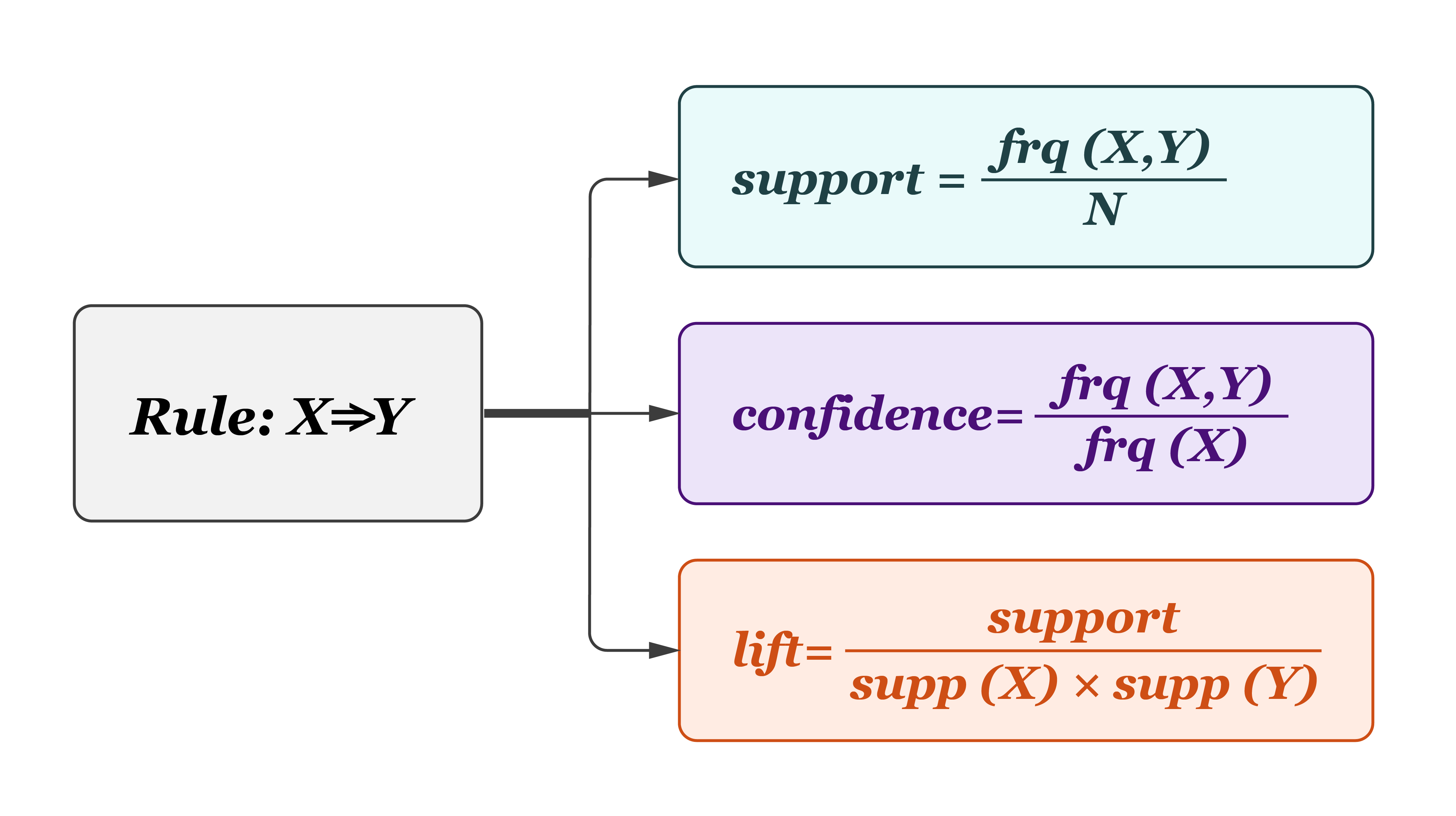Association rule learning is a rule-based learning method for discovering relations between variables in databases. It is intended to identify strong rules discovered in databases using some measures of interestingness.

Harp-DAAL support a Batch mode of Association Rules based on the Apriori algorithm 1.

## Covariance[Learn More]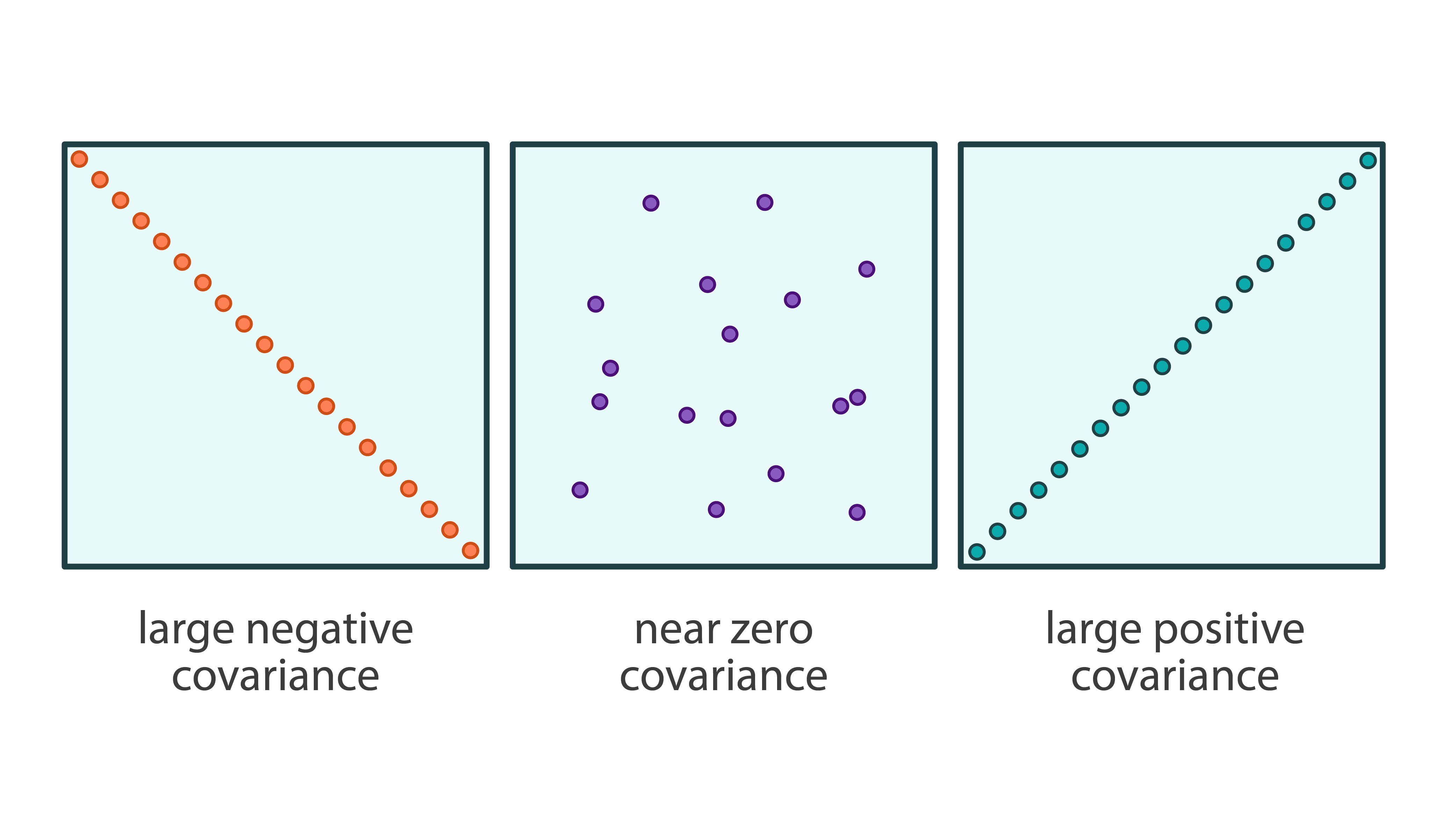Covariance, in probability theory and statistics, is a measure of the joint variability of two random variables. The sign of the covariance shows the tendency in the linear relationship between the variables. The correlation is the covariance normalized to be between -1 and +1. A positive correlation indicates the extent to which variables increase or decrease simultaneously. A negative correlation indicates the extent to which one variable increases while the other one decreases.

Harp-DAAL supports distributed modes of Covariance for both of dense and sparse (CSR format) input data.

## Boosting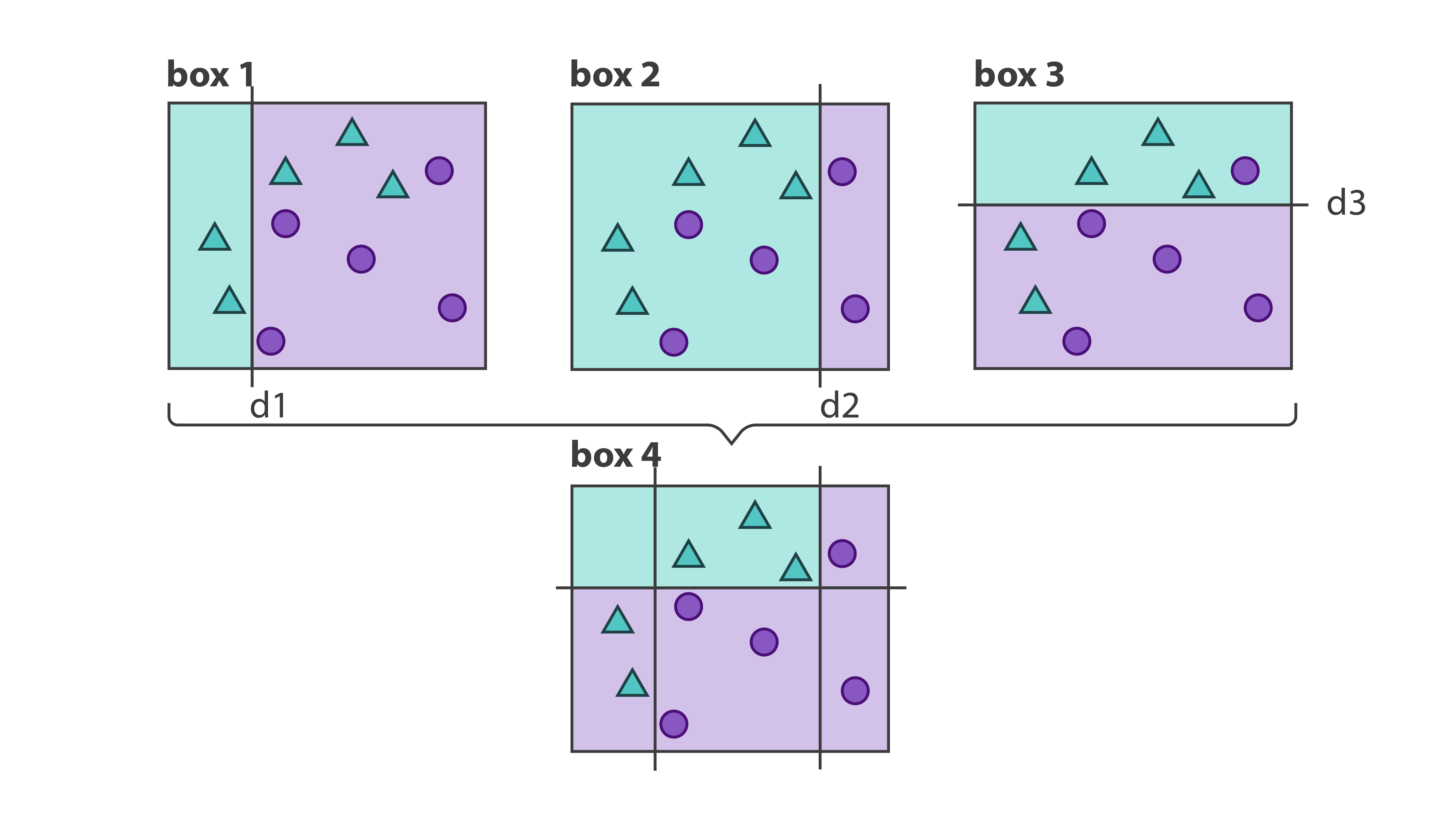Boosting is a group of algorithms aiming to construct a strong classifier from a set of weighted weak classifiers through iterative re-weighting based on accuracy measurement for weak classifiers. A weak classifier typically has only slightly better performance than random guessing, which are very simple, fast, and focusing on specific feature classification. Harp-DAAL supports batch modes of the following three boosting algorithms.

### AdaBoost Classifier [Learn More]

AdaBoost algorithm performs well on a variety of data sets except some noisy data 2. AdaBoost in Harp-DAAL is a binary classifier.

### BrownBoost Classifier [Learn More]

BrownBoost is a boosting classification algorithm. It is more robust to noisy data sets than other boosting classification algorithms. BrownBoost in Harp-DAAL is a binary classifier.

### LogitBoost Classifier [Learn More]

LogitBoost and AdaBoost are close to each other in the sense that both perform an additive logistic regression. The difference is that AdaBoost minimizes the exponential loss, whereas LogitBoost minimizes the logistic loss.

LogitBoost in Harp-DAAL is a multi-class classifier.

## Naive Bayes Classifier[Learn More]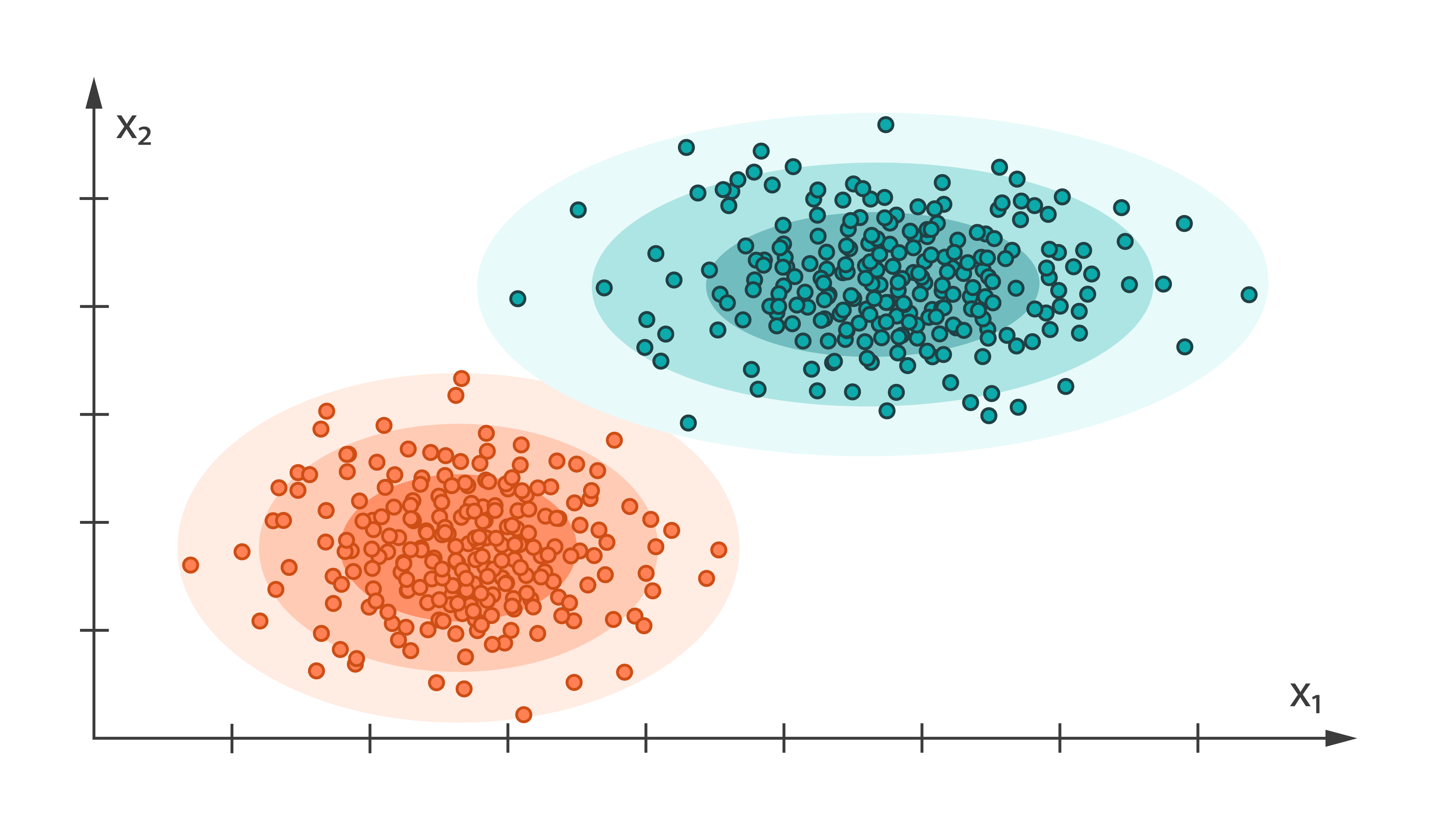Naïve Bayes is a set of simple and powerful classification methods often used for text classification, medical diagnosis, and other classification problems. In spite of their main assumption about independence between features, Naïve Bayes classifiers often work well when this assumption does not hold. An advantage of this method is that it requires only a small amount of training data to estimate model parameters.

Harp-DAAL currently supports distributed mode of Multinomial Naive Bayes 3 for both of dense and sparse (CSR format) input datasets.

## K-means Clustering[Learn More]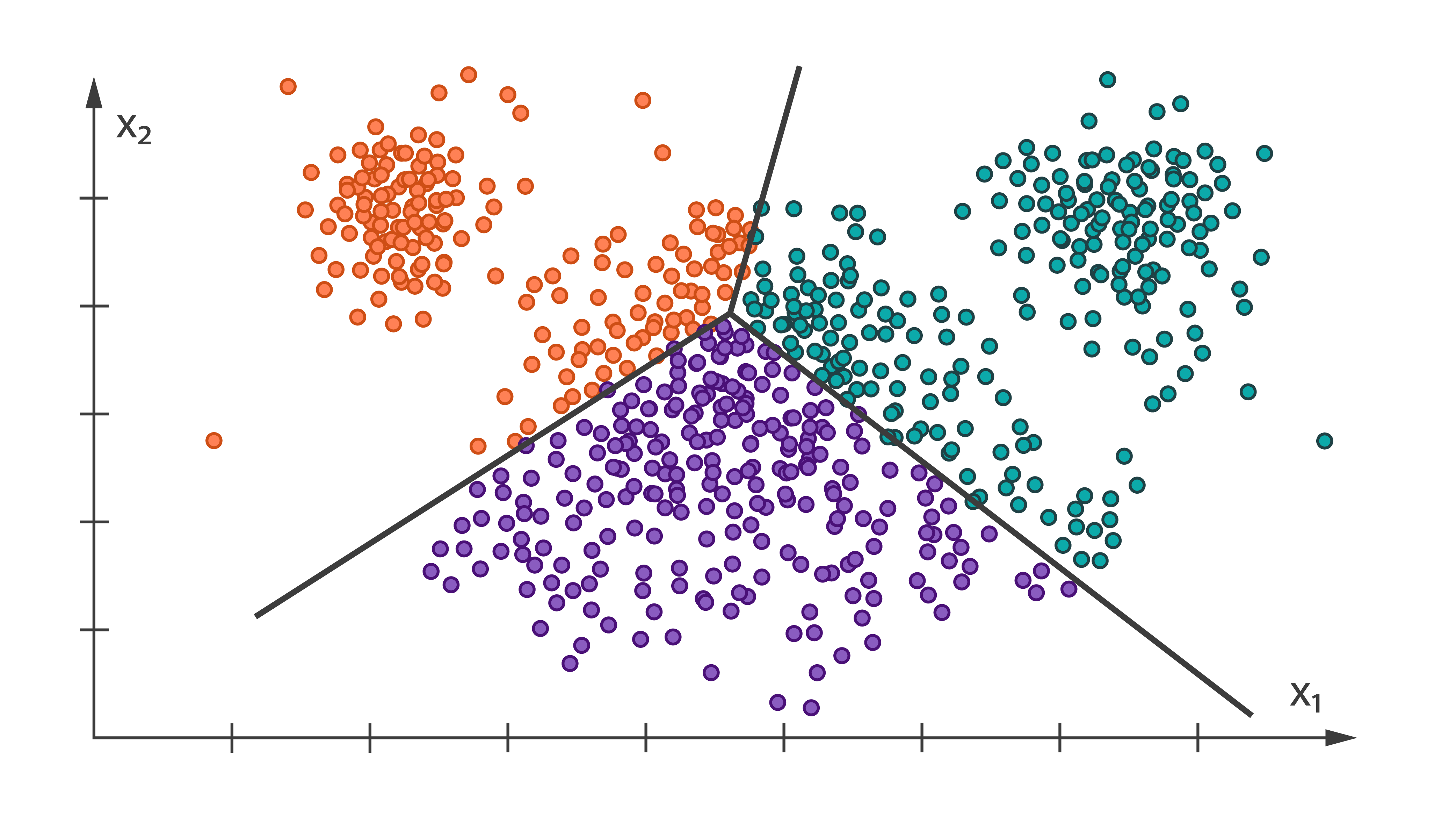K-means is a widely used clustering algorithm in machine learning community. It iteratively computes the distance between each training point to every centroids, re-assigns the training point to new cluster and re-compute the new centroid of each cluster. In other words, the clustering methods enable reducing the problem of analysis of the entire data set to the analysis of clusters.

Harp-DAAL currently supports distributed mode of K-means clustering for both of dense and sparse (CSR format) input datasets.

## Expectation Maximization[Learn More]Expectation-Maximization (EM) algorithm is an iterative method for finding the maximum likelihood and maximum a posteriori estimates of parameters in models that typically depend on hidden variables.

Harp-DAAL currently supports batch mode of EM 45 for dense input datasets.

## Principle Component Analysis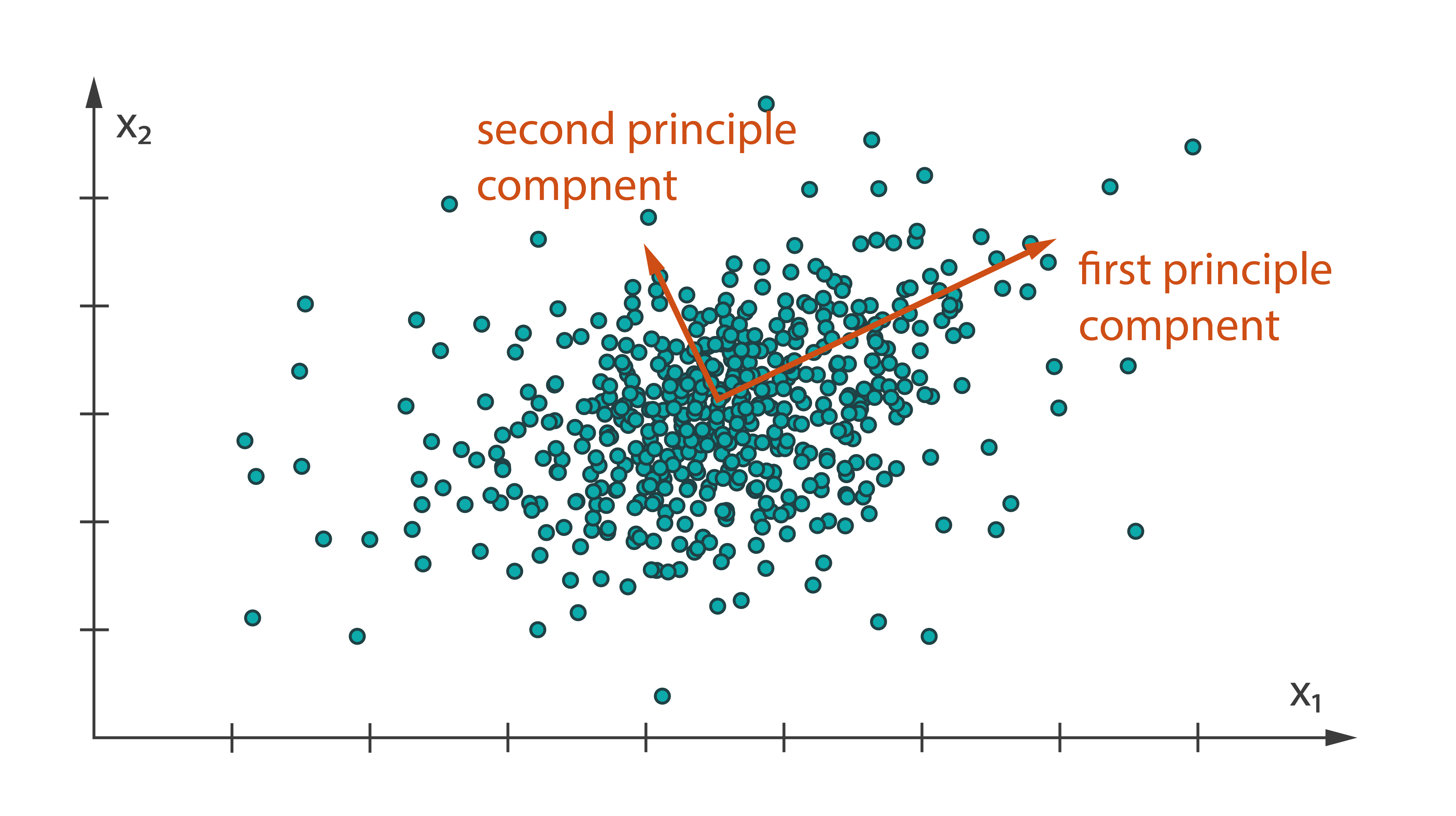Principle Component Analysis (PCA) is a widely used statistical procedure that uses an orthogonal transformation to convert a set of observations of possibly correlated variables into a set of values of linearly uncorrelated variables called principal components (or sometimes, principal modes of variation). PCA can be done by two methods:

• Eigenvalue decomposition of a data covariance (or cross-correlation)
• Singular Value Decomposition of a data

It is usually performed after normalizing (centering by the mean) the data matrix for each attribute.

In Harp-DAAL, we have two methods for computing PCA

### SVD Based PCA [Learn More]

The input is a set of p-dimensional dense vectors, DAAL kernel invokes a SVD decomposition to find out \$p_r\$ principle directions (Eigenvectors)

Harp-DAAL provides distributed mode for SVD based PCA for dense input datasets.

### Correleation Based PCA [Learn More]

The input is a \$p\tims p\$ correlation matrix, and the DAAL PCA kernel will find out the \$p_r\$ directions 6.

Harp-DAAL provides distributed mode for Correlation based PCA for both of dense and sparse (CSR format) input datasets.

## Single Value Decomposition[Learn More]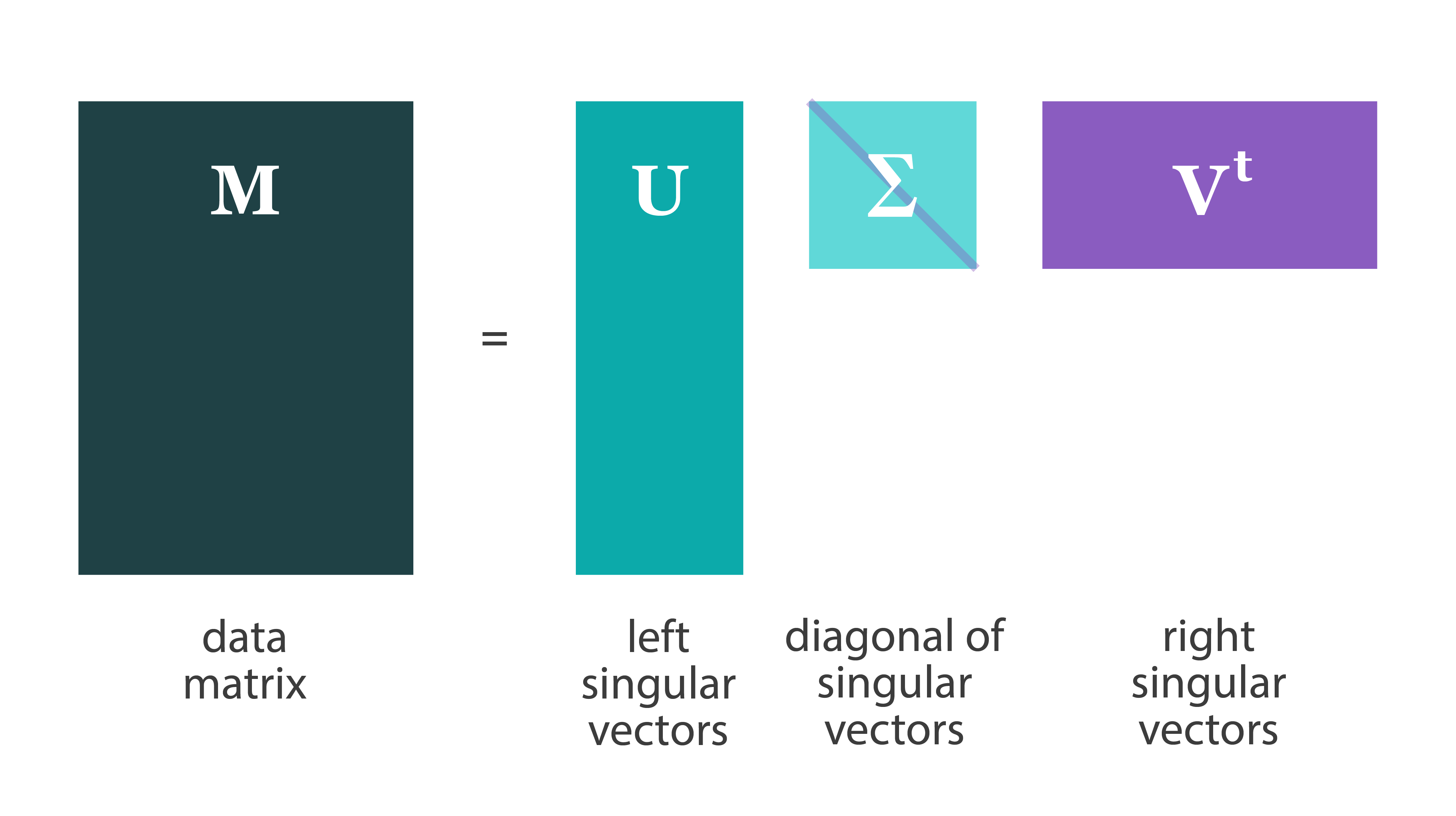Singular Value Decomposition is a method which seeks to reduce the rank of a data matrix, thus finding the unique vectors, features, or characteristics of the data matrix at hand. This algorithm has been used in, but is not limited to signal processing, weather prediction, hotspot detection, and recommender systems.

Harp-DAAL currently supports the distributed mode for dense input datasets

## QR Decomposition[Learn More]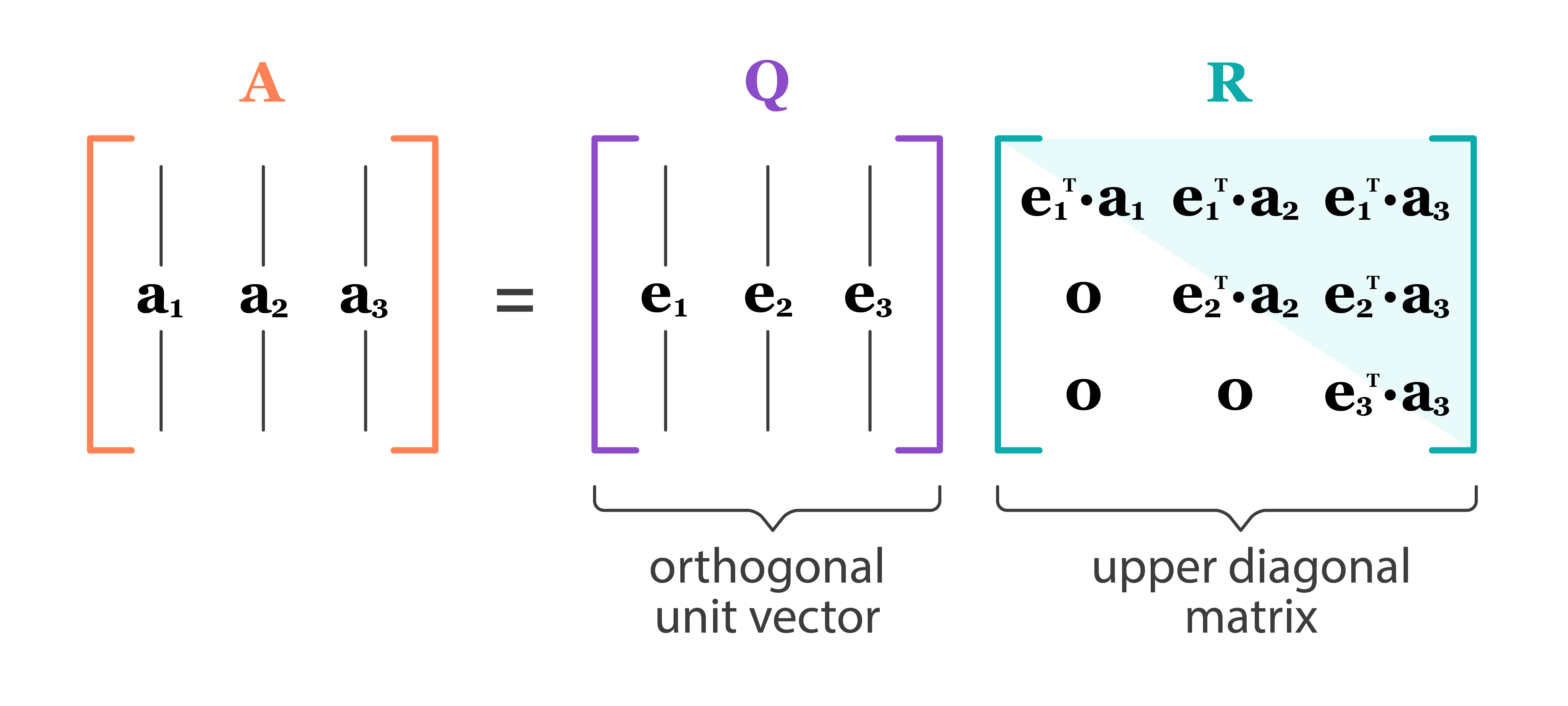The QR decomposition or QR factorization of a matrix is a decomposition of the matrix into an orthogonal matrix and a triangular matrix. A QR decomposition of a real square matrix A is a decomposition of A as A = QR, where Q is an orthogonal matrix (its columns are orthogonal unit vectors meaning QTQ = I) and R is an upper triangular matrix (also called right triangular matrix).

Harp-DAAL currently supports distributed mode of QR for dense input datasets.

## Pivoted QR Decomposition[Learn More]

QR decomposition with column pivoting introduces a permutation matrix P and convert the original A=QR to AP=QR. Column pivoting is useful when A is (nearly) rank deficient, or is suspected of being so. It can also improve numerical accuracy.

Harp-DAAL currently supports distributed mode of Pivoted QR for dense input datasets.

## Cholesky Decomposition[Learn More]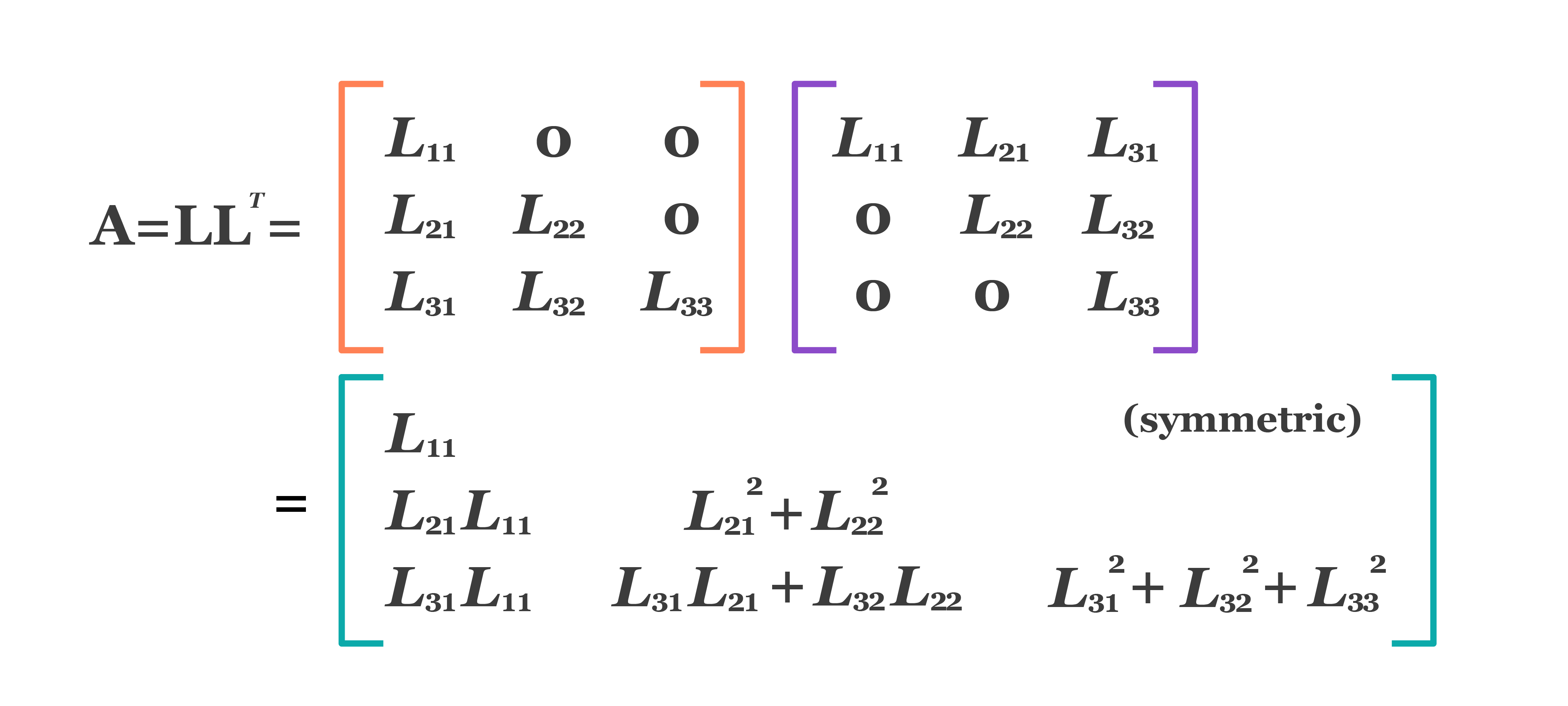Cholesky decomposition is a matrix factorization technique that decomposes a symmetric positive-definite matrix into a product of a lower triangular matrix and its conjugate transpose.

Harp-DAAL currently supports batch mode of Cholesky for dense input datasets.

## Moments of Low Order[Learn More]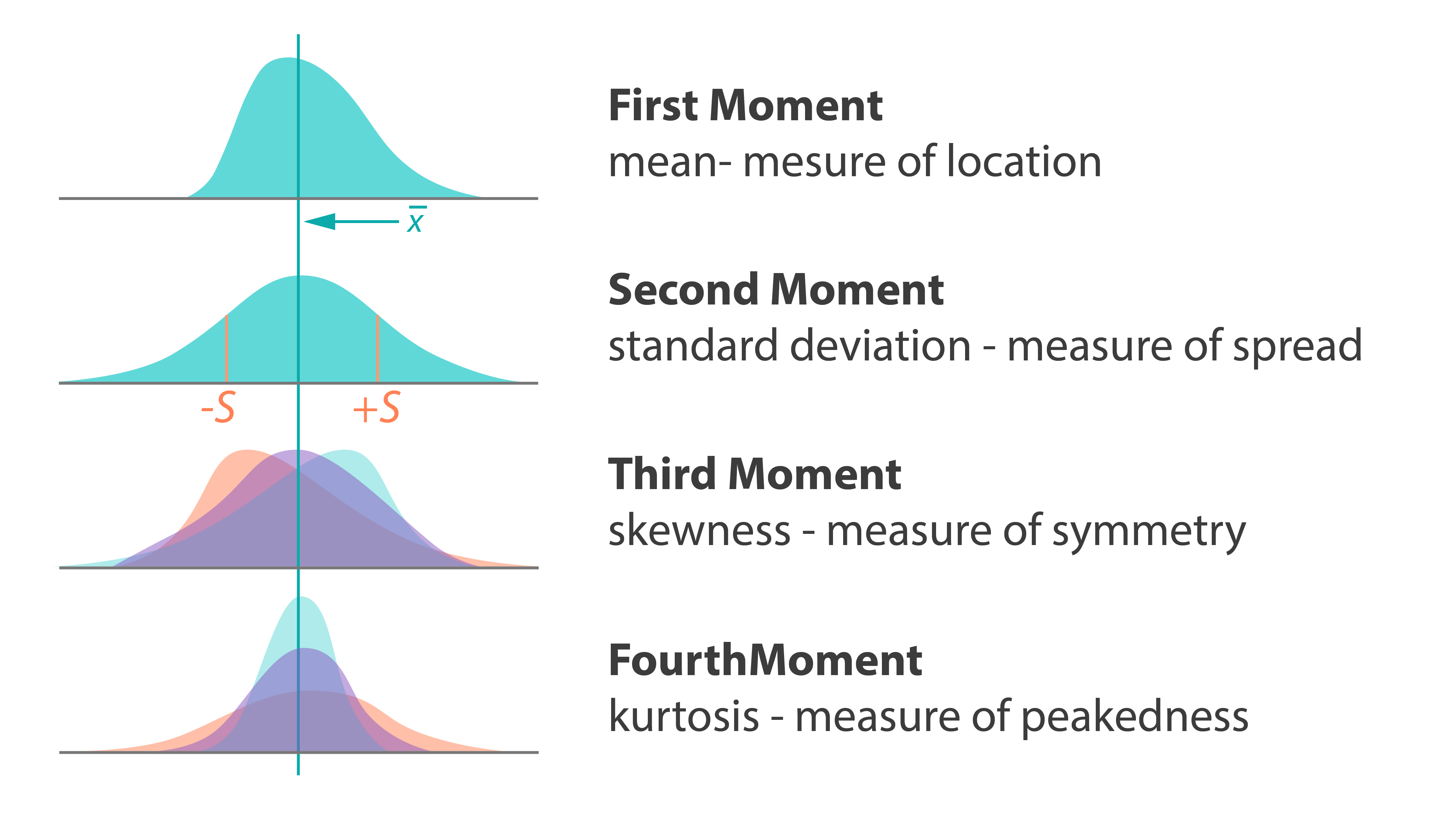Moments are basic quantitative measures of data set characteristics such as location and dispersion. We compute the following low order characteristics: minimums/maximums, sums, means, sums of squares, sums of squared differences from the means, second order raw moments, variances, standard deviations, and variations.

Harp-DAAL supports the distributed mode of Low-order moments for both of dense and sparse (CSR format) input datasets

## Outlier Detection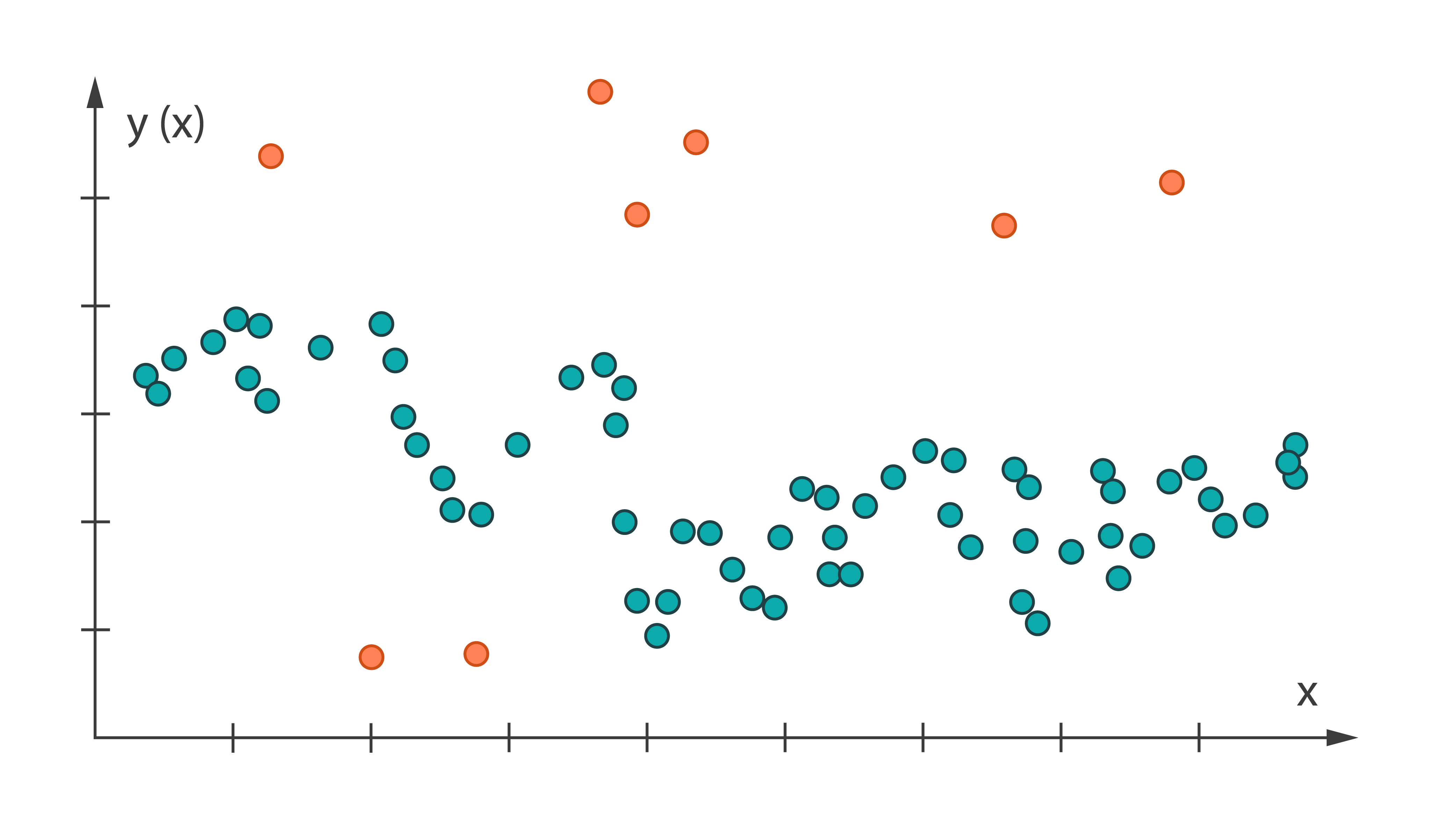Outlier detection methods aim to identify observation points that are abnormally distant from other observation points.

### Univariate [Learn More]

A univariate outlier is an occurrence of an abnormal value within a single observation point.

### Multivariate [Learn More]

In multivariate outlier detection methods, the observation point is the entire feature vector.

Harp-DAAL currently supports batch mode of outlier detection for dense input datasets.

## Sorting[Learn More]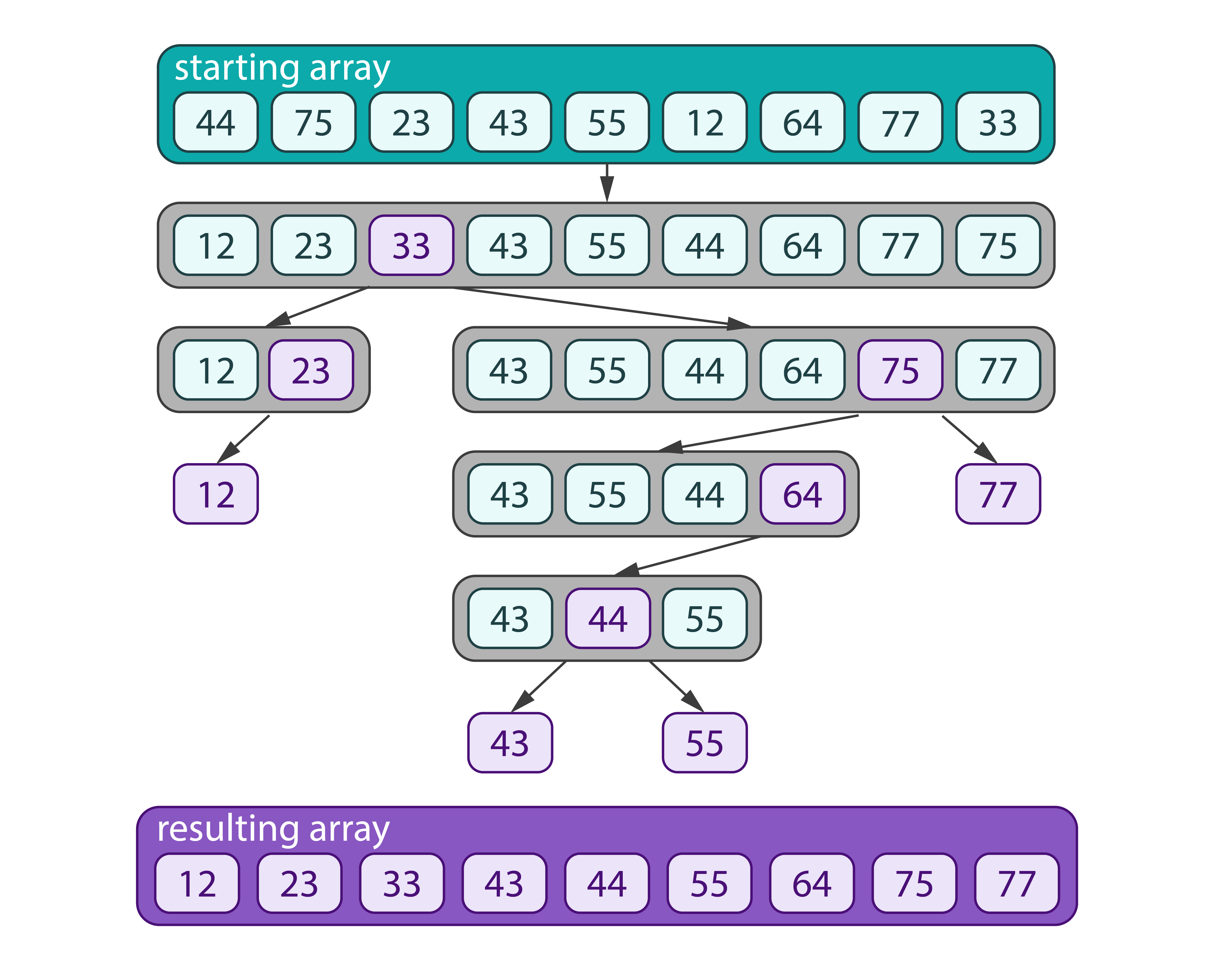Sorting is an algorithm to sort the observations by each feature (column) in the ascending order.

Harp-DAAL currently supports batch mode of sorting for dense input datasets.

## Quantile[Learn More]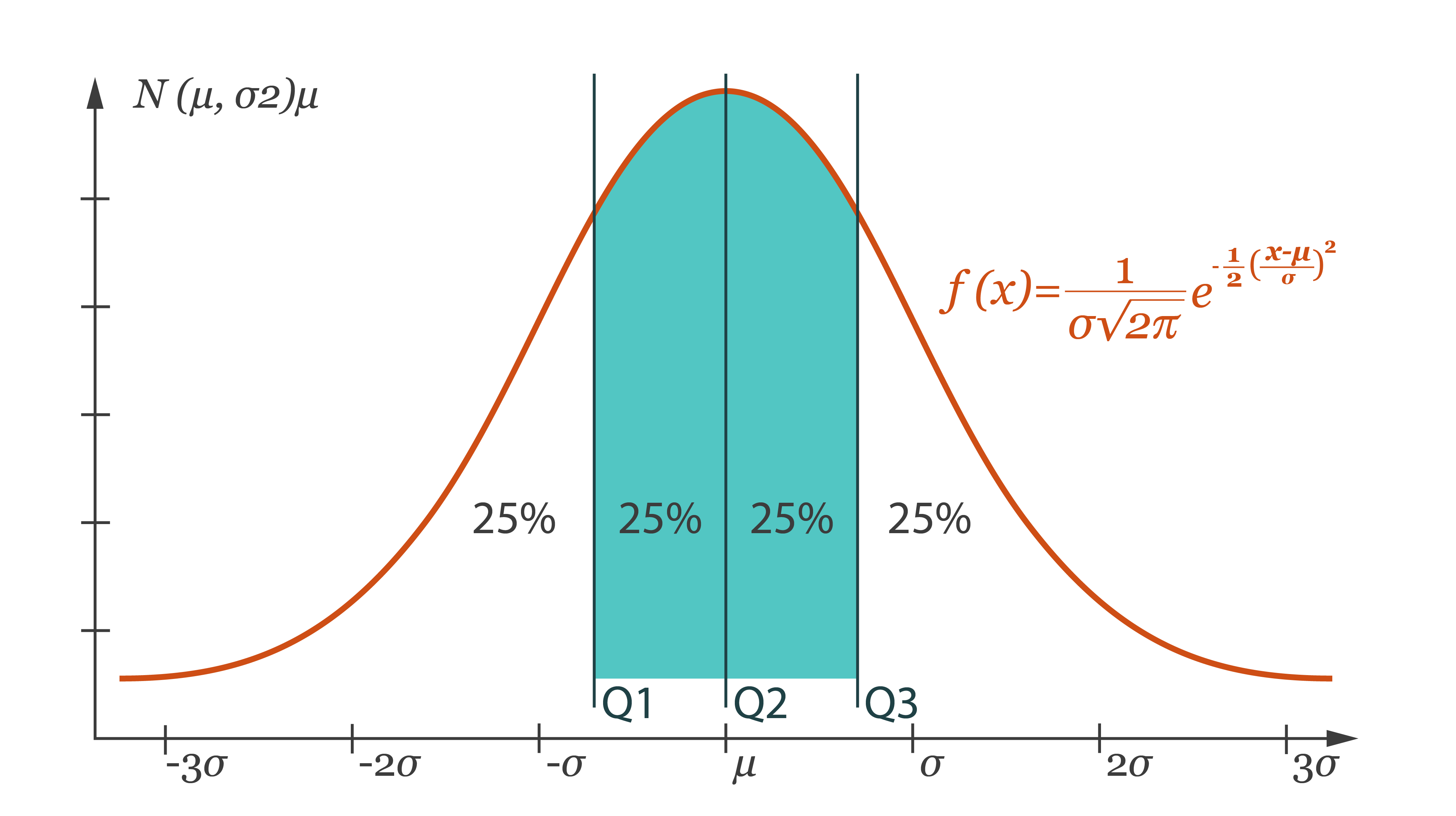Quantile is an algorithm to analyze the distribution of observations. Quantiles are the values that divide the distribution so that a given portion of observations is below the quantile.

Harp-DAAL currently supports batch mode of Quantile for dense input datasets.

## Quality Metrics[Learn More]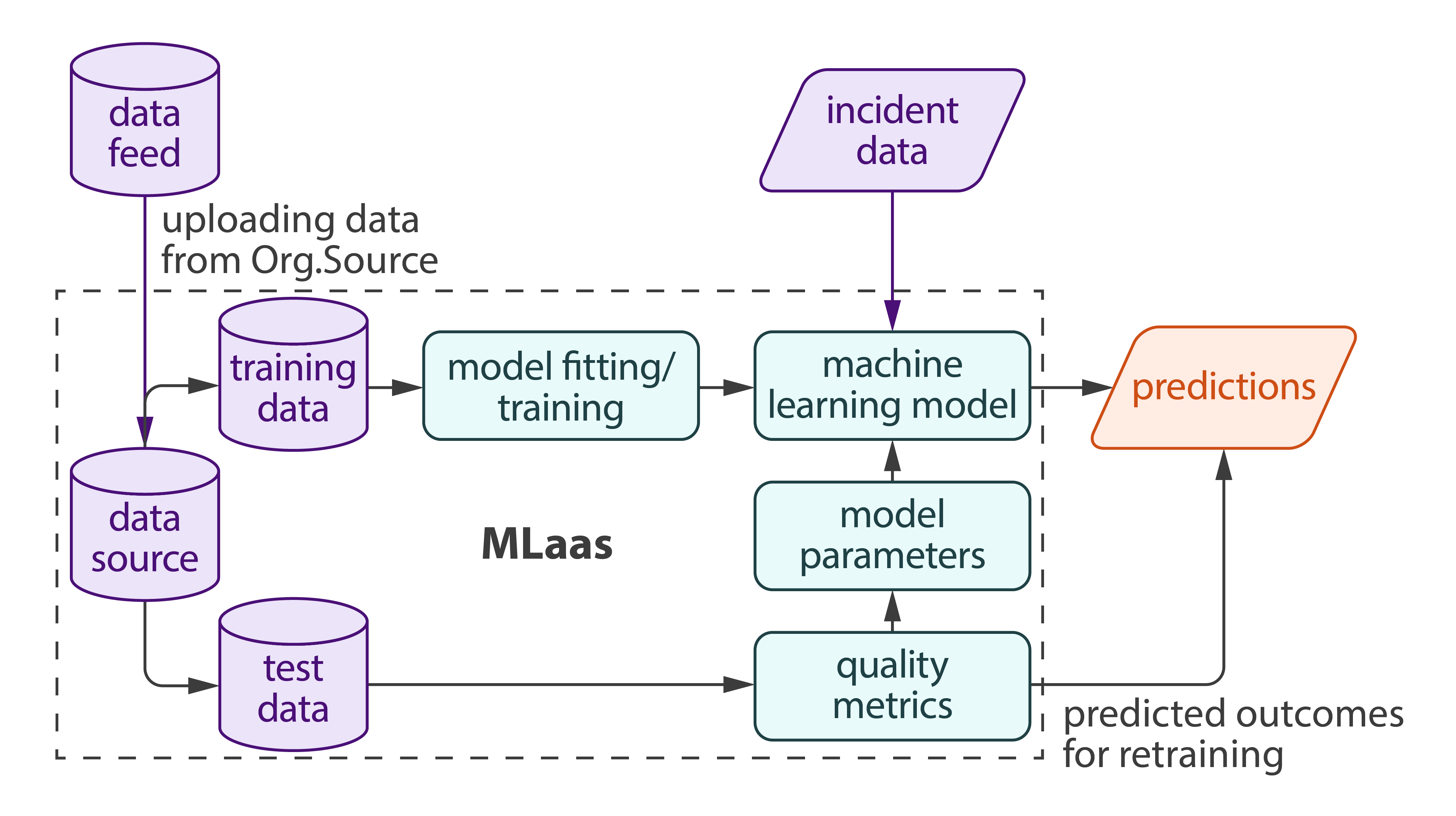A quality metric is a numerical characteristic or a set of connected numerical characteristics that represents the qualitative aspect of the result returned by an algorithm: a computed statistical estimate, model, or result of decision making.

Harp-DAAL currently supports batch mode of sorting for dense input datasets.

## Optimization Solvers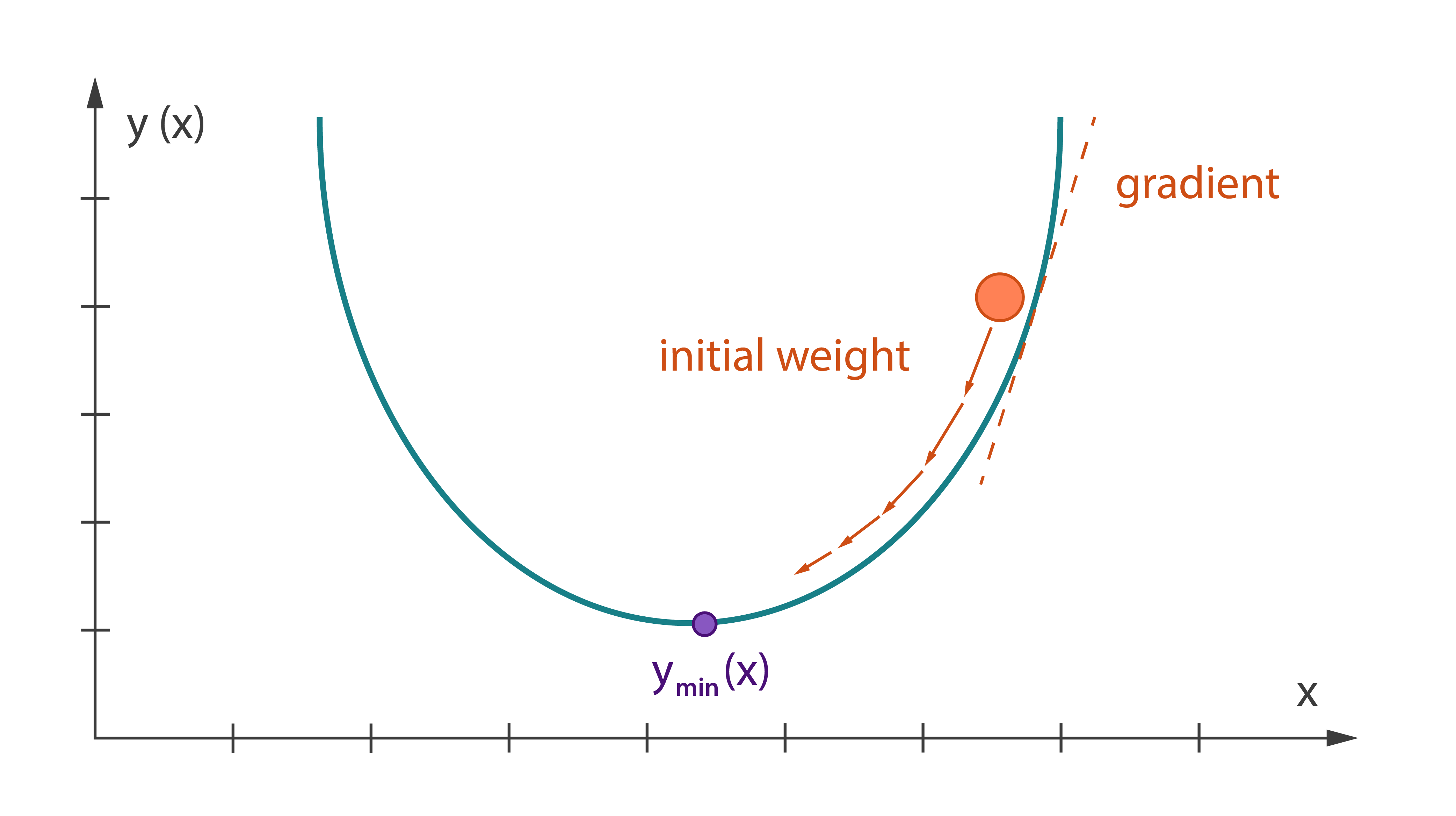An optimization solver is an algorithm to solve an optimization problem, which is to find the maximum or minimum of an objective function in the presence of constraints on its variables.

Harp-DAAL currently provides the following iterative optimization solvers

### Adaptive Subgradient Method [Learn More]

The adaptive subgradient method (AdaGrad) is from 7.

### Limited-Memory Broyden-Fletcher-Goldfarb-Shanno Algorithm [Learn More]

The limited-memory Broyden-Fletcher-Goldfarb-Shanno (LBFGS) algorithm is from 8.

### Stochastic Gradient Descent Algorithm [Learn More]

The stochastic gradient descent (SGD) algorithm is a special case of an iterative solver. The following computation methods are available in Intel DAAL for the stochastic gradient descent algorithm:

#### Mini-batch.

The mini-batch method (miniBatch) of the stochastic gradient descent algorithm is from 9.

#### Momentum

The momentum method (momentum) of the stochastic gradient descent algorithm is from 10.

## Normalization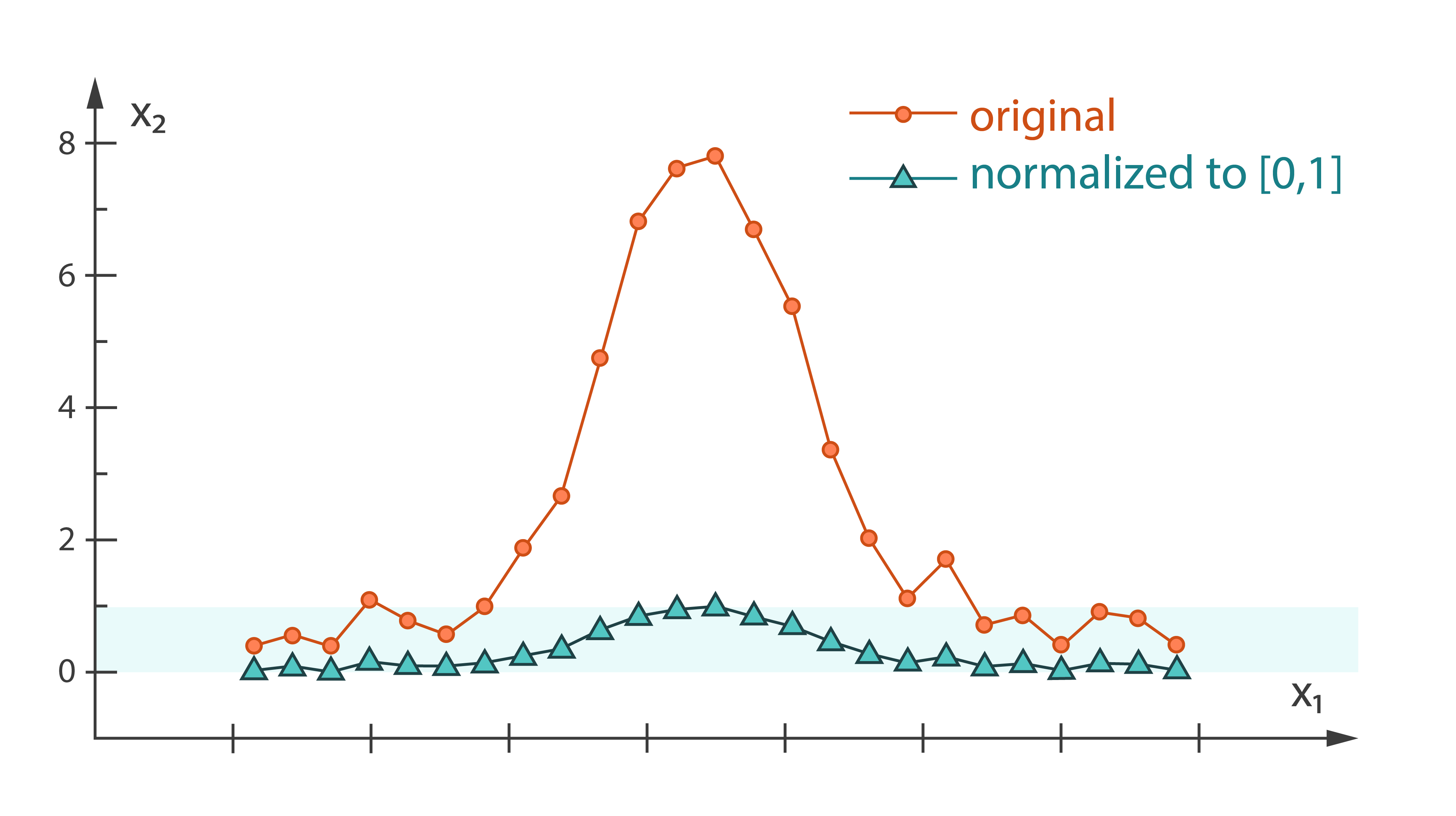In statistics and applications of statistics, normalization can have a range of meanings. In the simplest cases, normalization of ratings means adjusting values measured on different scales to a notionally common scale, often prior to averaging. In more complicated cases, normalization may refer to more sophisticated adjustments where the intention is to bring the entire probability distributions of adjusted values into alignment.

Harp-DAAL currently supports batch mode of normalization for dense input datasets, and it provides two algorithm kernels.

### Min-max [Learn More]

Min-max normalization is an algorithm to linearly scale the observations by each feature (column) into the range [a, b].

### Z-score [Learn More]

Z-score normalization is an algorithm to normalize the observations by each feature (column).

## Support Vector Machine Classifier[Learn More]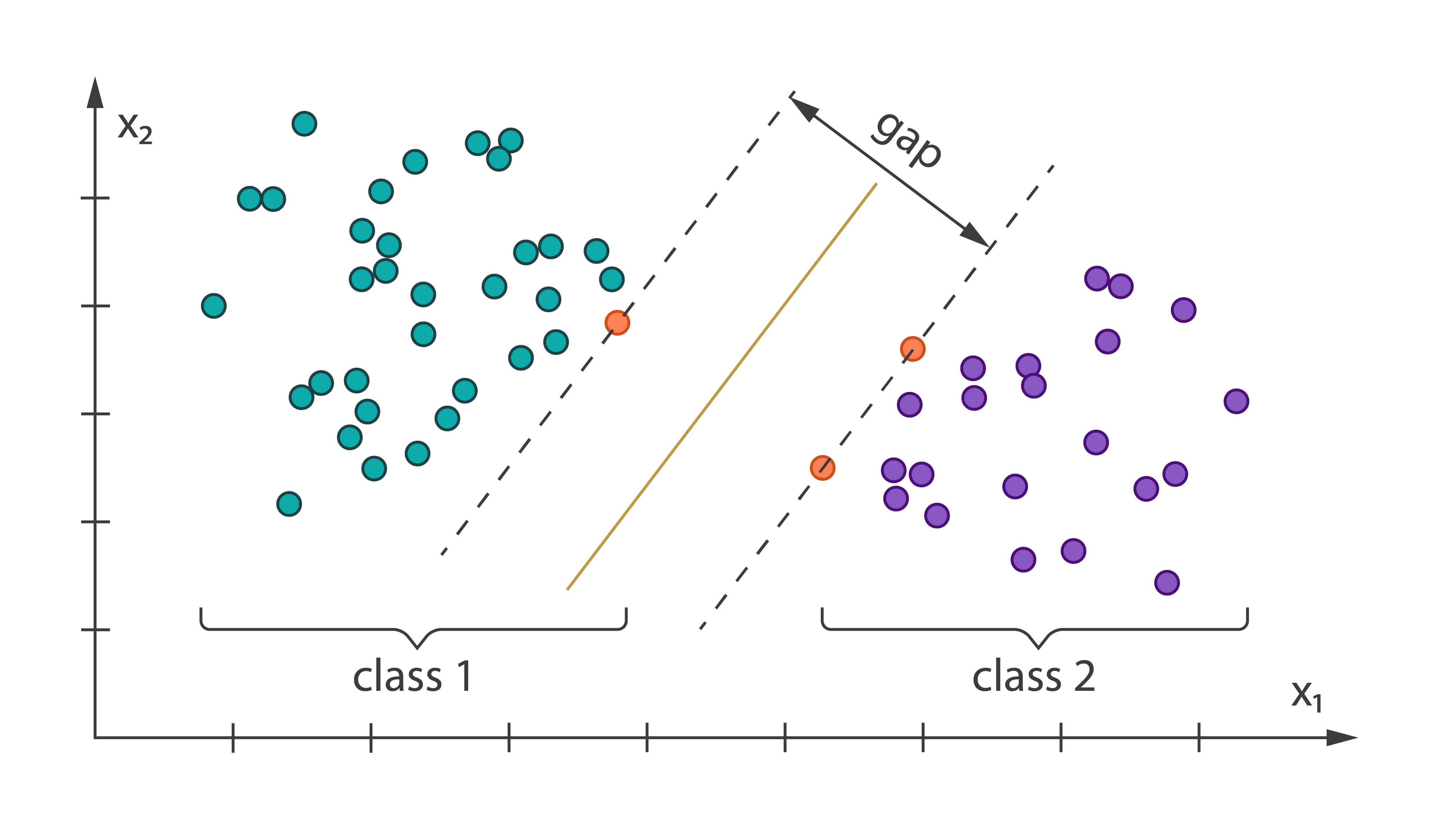Support Vector Machine (SVM) is among popular classification algorithms. It belongs to a family of generalized linear classification problems. Because SVM covers binary classification problems only in the multi-class case, SVM must be used in conjunction with multi-class classifier methods.

Harp-DAAL currently supports batch mode of multi-class SVM 11 for both of dense and sparse (CSR format) input datasets.

## K-Nearest Neighbors Classifier[Learn More]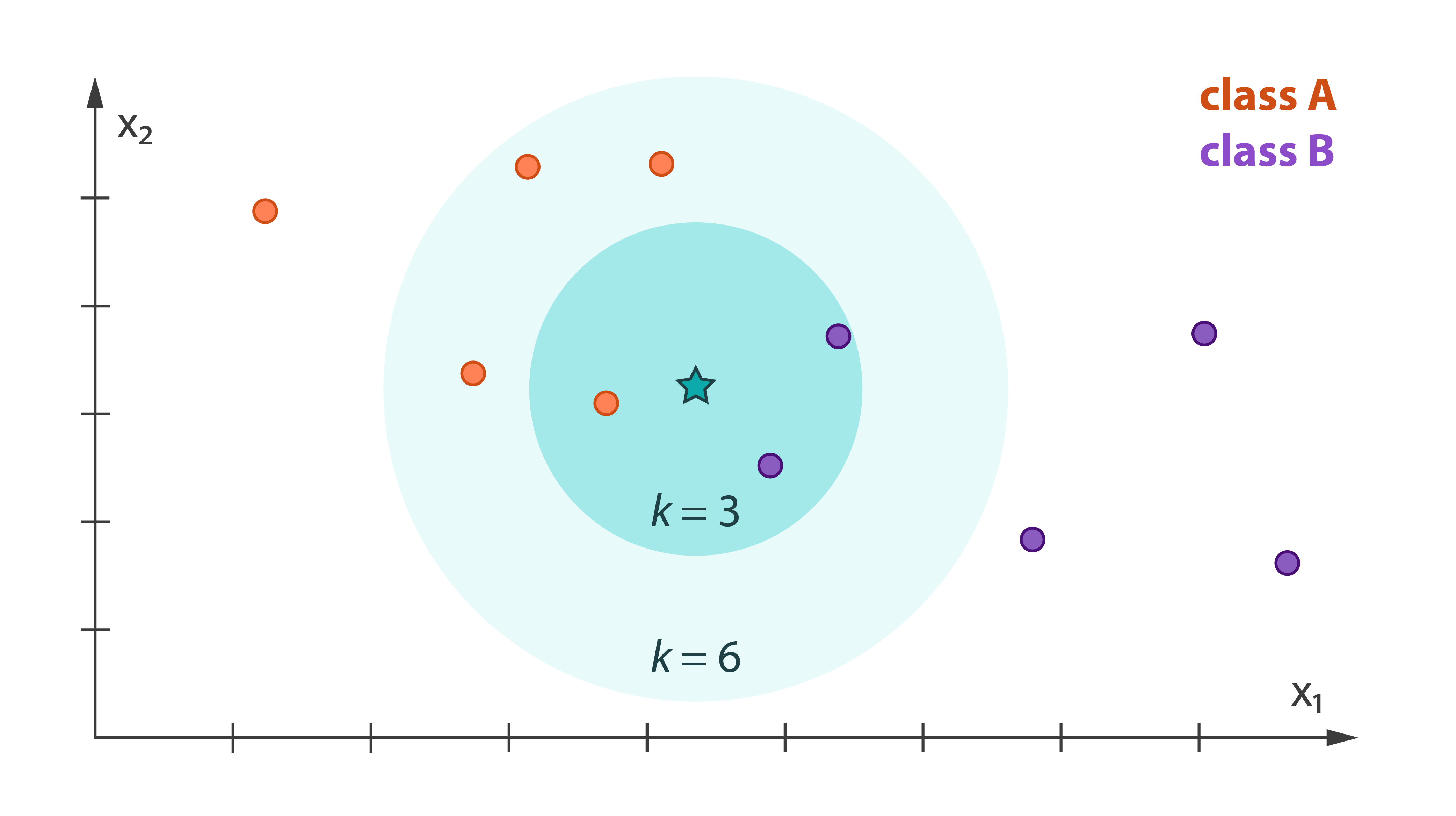k-Nearest Neighbors (kNN) classification is a non-parametric classification algorithm. The model of the kNN classifier is based on feature vectors and class labels from the training data set. This classifier induces the class of the query vector from the labels of the feature vectors in the training data set to which the query vector is similar. A similarity between feature vectors is determined by the type of distance (for example, Euclidian) in a multidimensional feature space.

Harp-DAAL currently supports batch mode of K-NN 1213 for dense input datasets.

## Decision Tree[Learn More]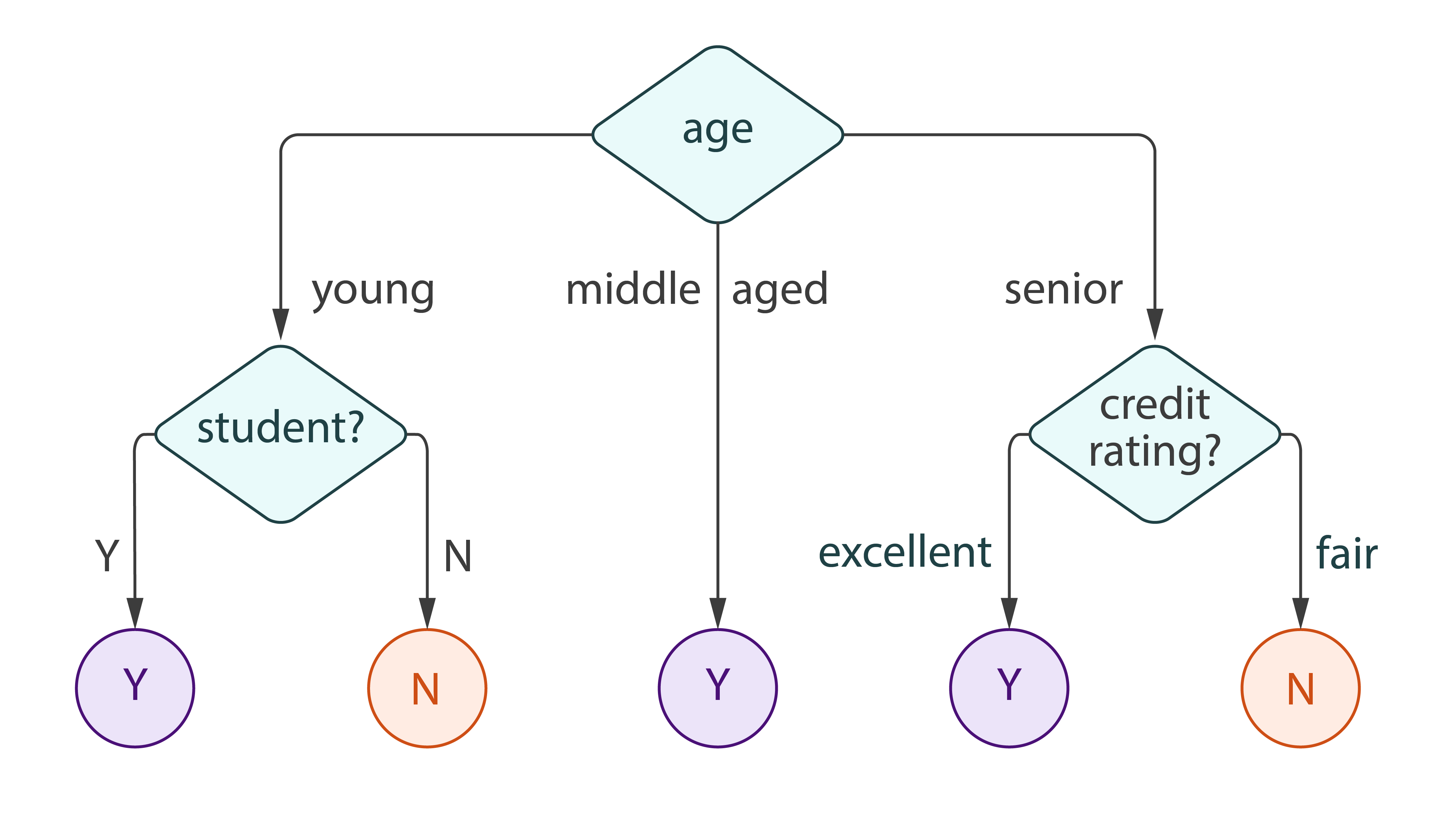Decision trees partition the feature space into a set of hypercubes, and then fit a simple model in each hypercube. The simple model can be a prediction model, which ignores all predictors and predicts the majority (most frequent) class (or the mean of a dependent variable for regression), also known as 0-R or constant classifier.

Harp-DAAL currently supports batch mode of Decision Tree for dense input datasets, which includes two sub-types

• Classification
• Regression

## Decision Forest[Learn More]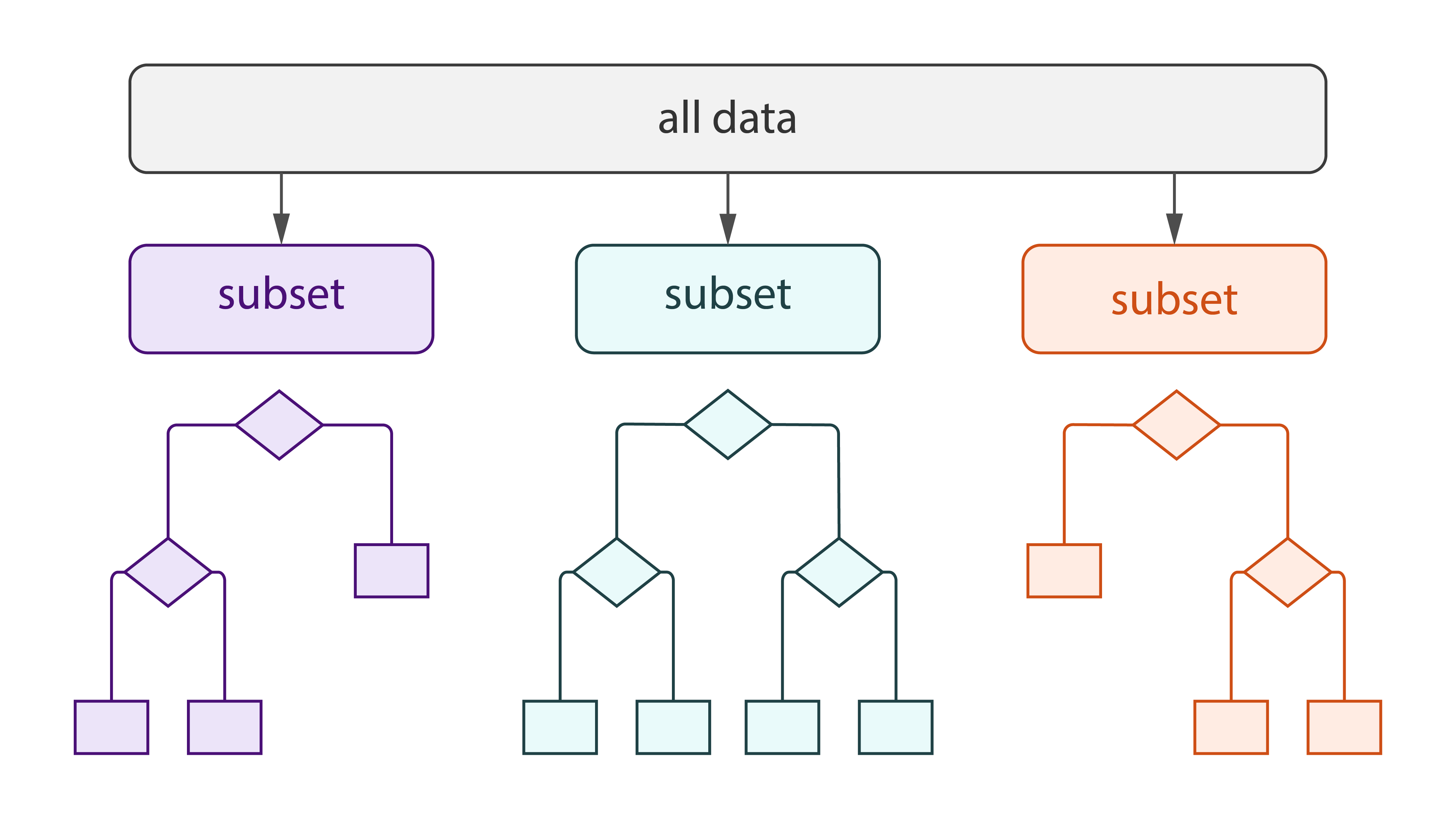Decision forest classification and regression algorithms are based on an ensemble of tree-structured classifiers (decision trees) built using the general technique of bootstrap aggregation (bagging) and random choice of features.

Harp-DAAL currently supports batch mode of Decision Forest for dense input datasets, which includes two sub-types

• Classification
• Regression

## Kernel Functions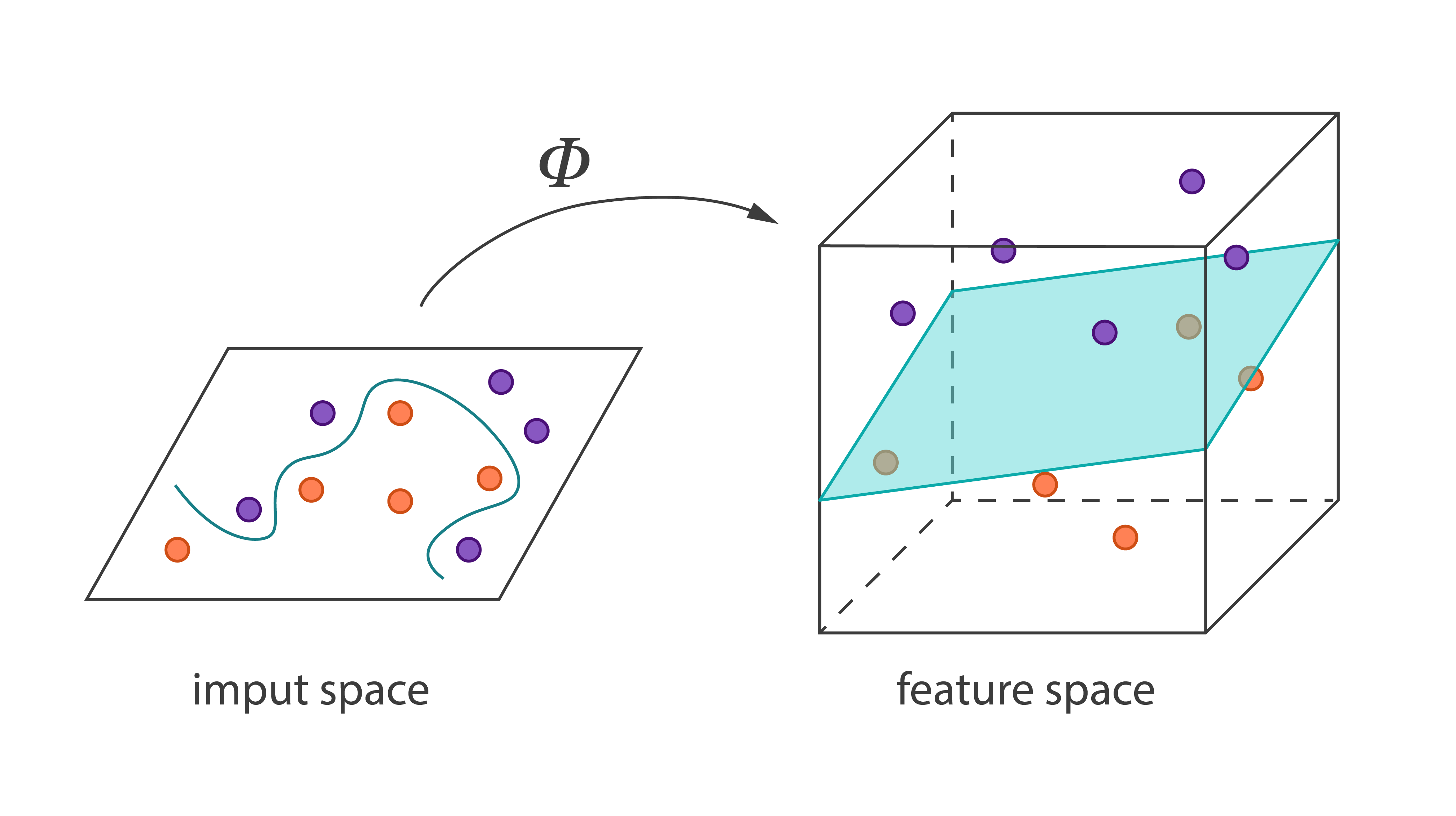Kernel functions form a class of algorithms for pattern analysis. The main characteristic of kernel functions is a distinct approach to this problem. Instead of reducing the dimension of the original data, kernel functions map the data into higher-dimensional spaces in order to make the data more easily separable there.

Harp-DAAL currently supports batch mode of kernel functions for bothh of dense and sparse (CSR format) input datasets, which includes two sub-types

### Linear Kernel [Learn More]

A linear kernel is the simplest kernel function.

### Radial Basis Function Kernel [Learn More]

The Radial Basis Function (RBF) kernel is a popular kernel function used in kernelized learning algorithms.

## Stump Weak Learner Classifier[Learn More]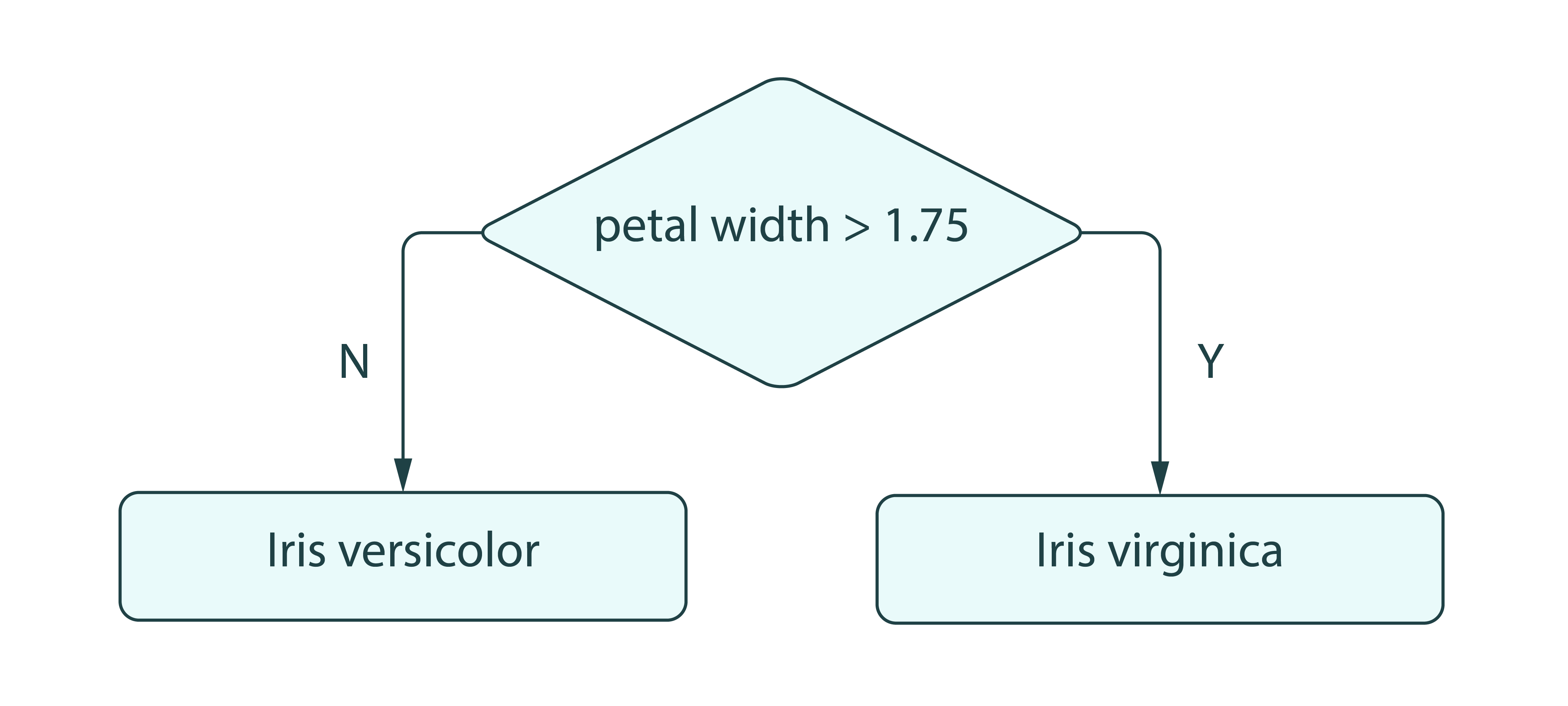A decision stump is a model that consists of a one-level decision tree 14 where the root is connected to terminal nodes (leaves).

Harp-DAAL currently supports batch mode of Stump for dense input datasets.

## Linear Regression[Learn More]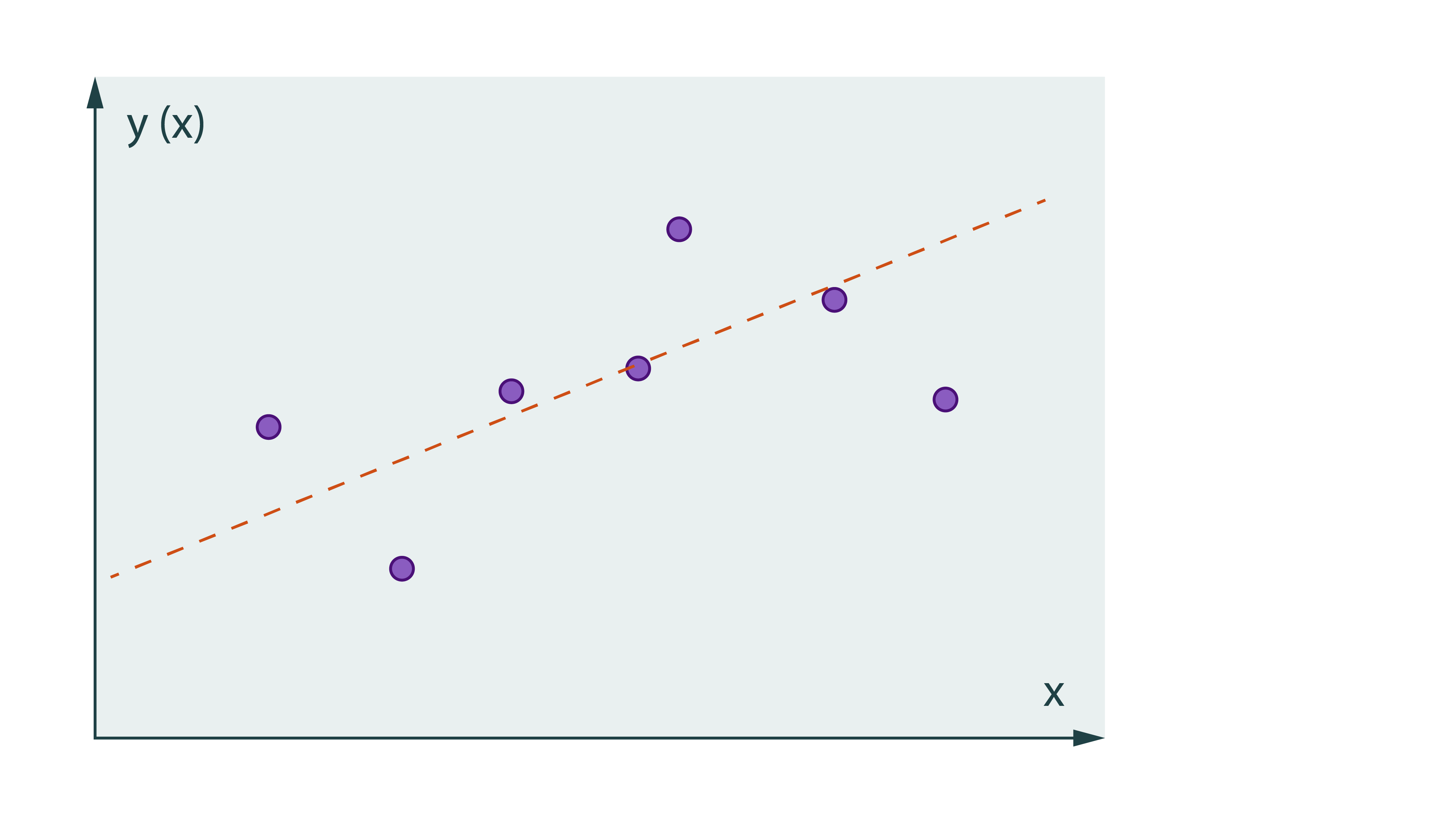In statistics, linear regression is an approach for modelling the relationship between a scalar dependent variable y and one or more explanatory variables (or independent variables) denoted X. In linear regression, the relationships are modeled using linear predictor functions whose unknown model parameters are estimated from the data. Such models are called linear models.

Harp-DAAL currently supports distributed mode of linear regression for dense input datasets. It has two algorithmic variants 15:

• Linear regression through normal equation
• Linear regression through QR decomposition.

## Ridge Regression[Learn More]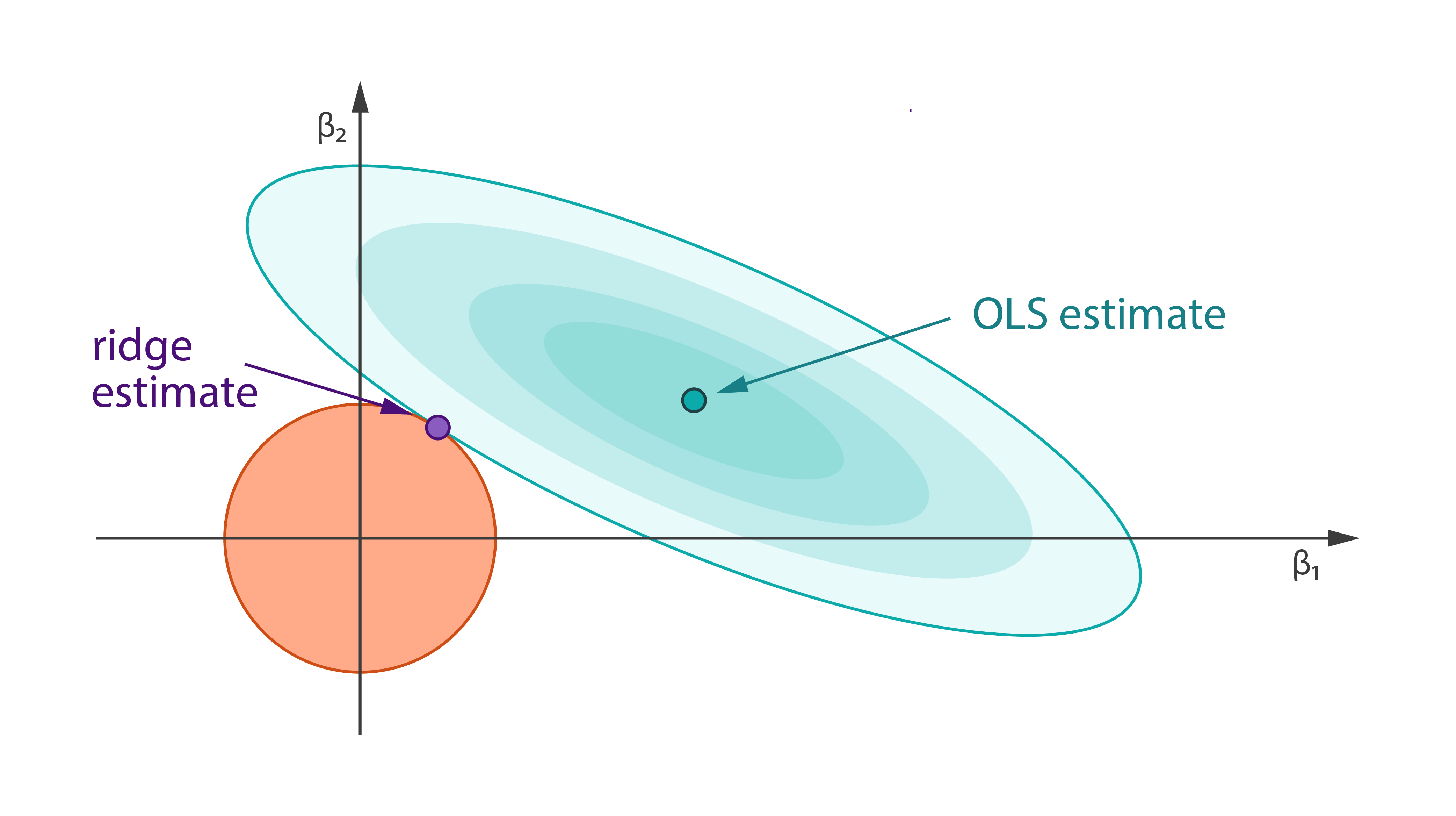Ridge Regression is a technique for analyzing multiple regression data that suffer from multicollinearity. When multicollinearity occurs, least squares estimates are unbiased, but their variances are large so they may be far from the true value. By adding a degree of bias to the regression estimates, ridge regression reduces the standard errors. It is hoped that the net effect will be to give estimates that are more reliable.

Harp-DAAL currently supports distributed mode of Ridge regression 16 for dense input datasets.

## Implicit Alternating Least Squares[Learn More]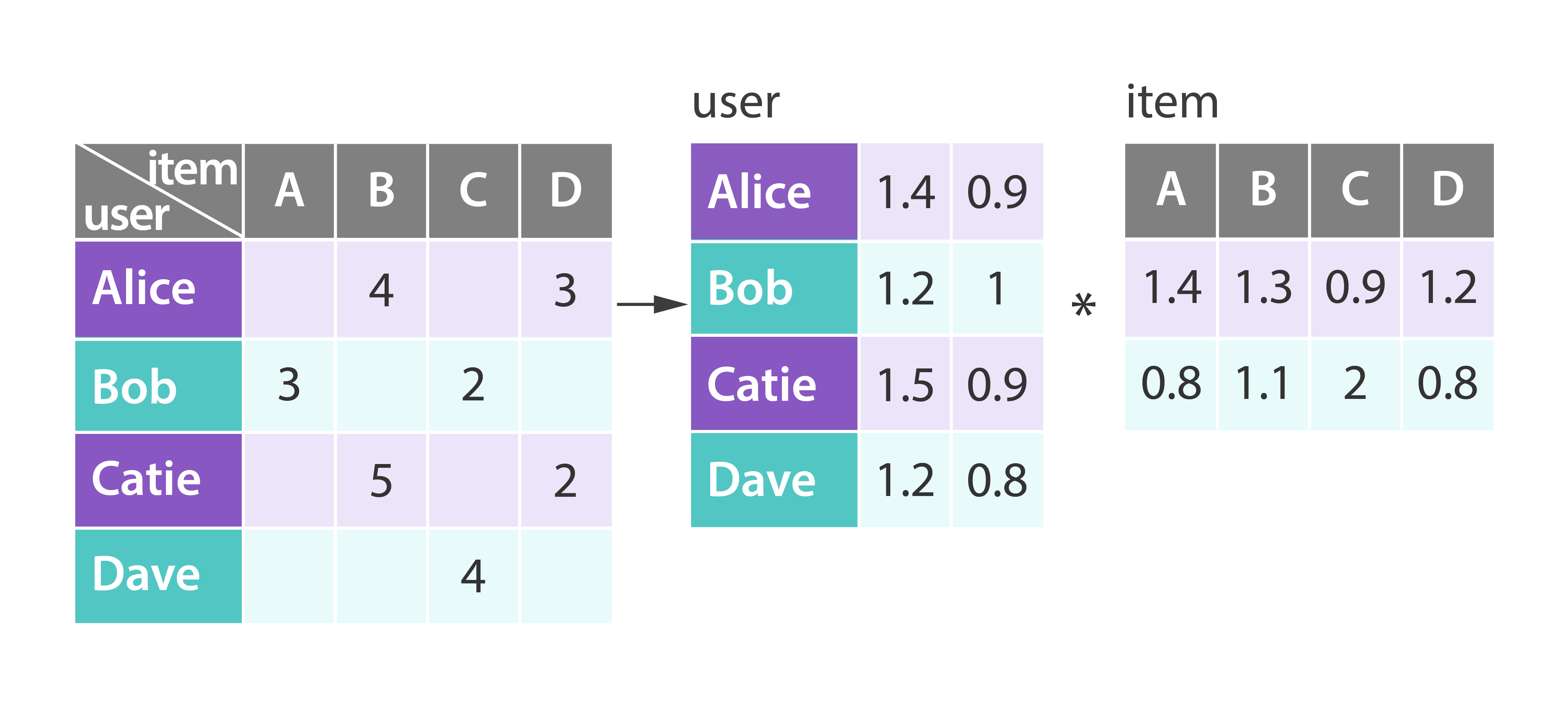Alternating least squares (ALS) is an algorithm used in recommender systems, which trains the model data X and Y to minimize the cost function as below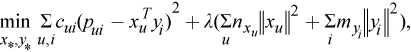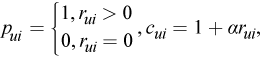where

• c_ui measures the confidence in observing p_ui
• alpha is the rate of confidence
• r_ui is the element of the matrix R
• labmda is the parameter of the regularization
• n_xu, m_yi denote the number of ratings of user u and item i respectively.

ALS alternatively computes model x and y independently of each other in the following formula: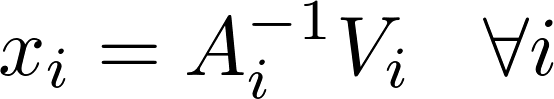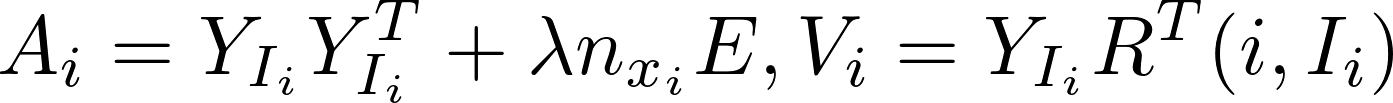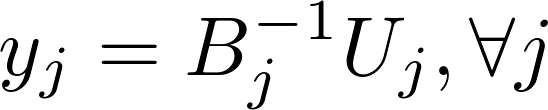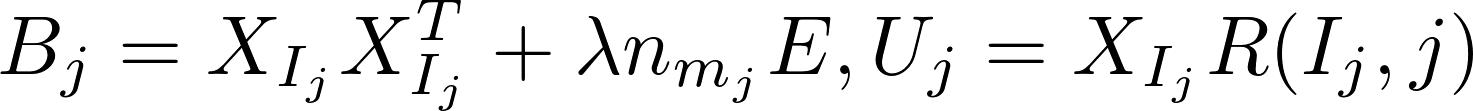Harp-DAAL currently supports distributed mode of ALS 1718 for dense and sparse (CSR format) input datasets.

## Neural Networks[Learn More]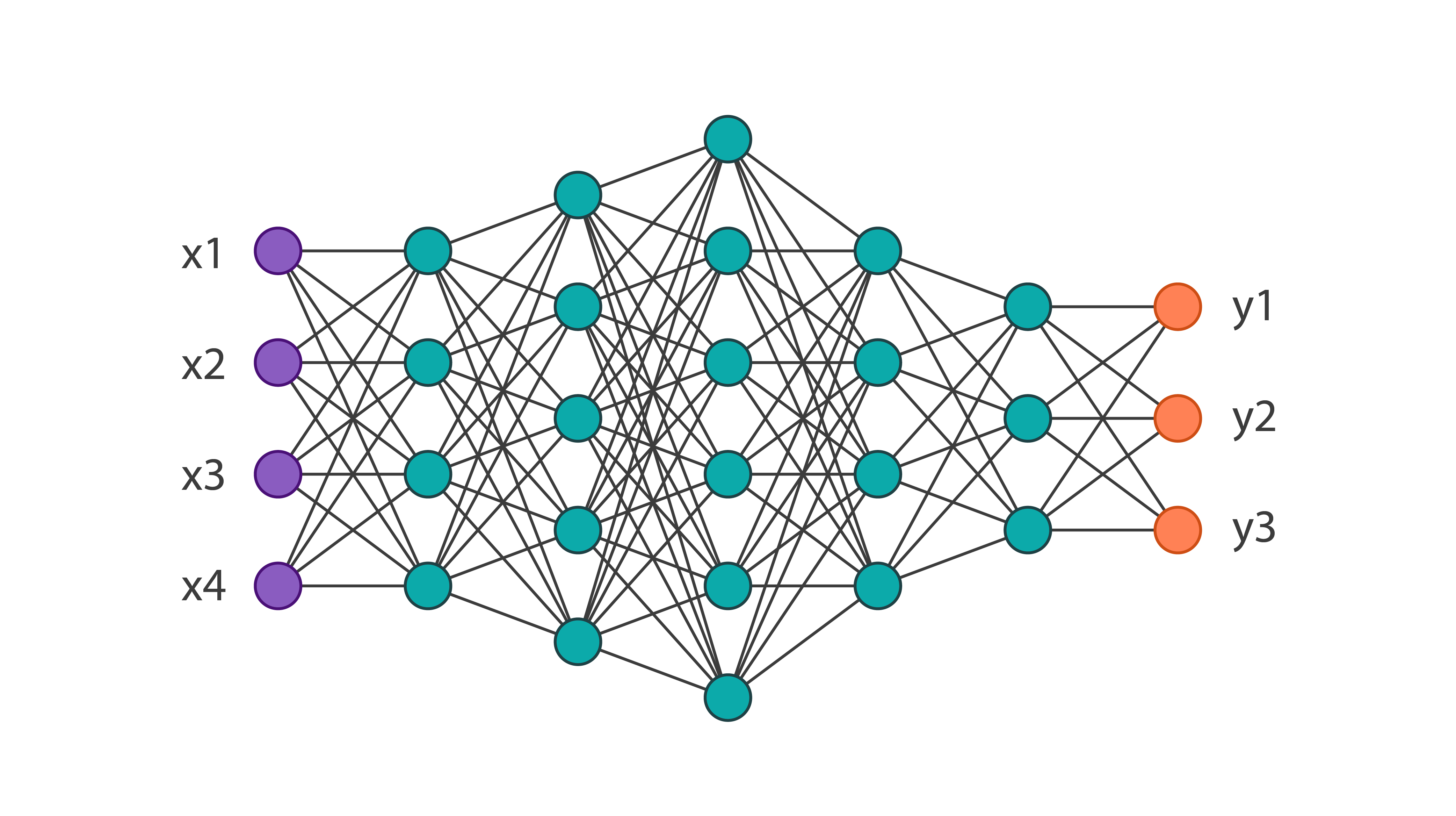Neural Networks are a beautiful biologically-inspired programming paradigm which enable a computer to learn from observational data. The motivation for the development of neural network technology stemmed from the desire to develop an artificial system that could perform “intelligent” tasks similar to those performed by the human brain. Neural networks, with their remarkable ability to derive meaning from complicated or imprecise data, can be used to extract patterns and detect trends that are too complex to be noticed by either humans or other computer techniques.

Harp-DAAL currently supports distributed mode of Neural Networks for dense input datasets.

1. Rakesh Agrawal, Ramakrishnan Srikant. Fast Algorithms for Mining Association Rules. Proceedings of the 20th VLDB Conference Santiago, Chile, 1994. [return]
2. Yoav Freund, Robert E. Schapire. Additive Logistic regression: a statistical view of boosting. Journal of Japanese Society for Artificial Intelligence (14(5)), 771-780, 1999. [return]
3. Jason D.M. Rennie, Lawrence, Shih, Jaime Teevan, David R. Karget. Tackling the Poor Assumptions of Naïve Bayes Text classifiers. Proceedings of the Twentieth International Conference on Machine Learning (ICML-2003), Washington DC, 2003. [return]
4. A.P.Dempster, N.M. Laird, and D.B. Rubin. Maximum-likelihood from incomplete data via the em algorithm. J. Royal Statist. Soc. Ser. B., 39, 1977. [return]
5. Trevor Hastie, Robert Tibshirani, Jerome Friedman. The Elements of Statistical Learning: Data Mining, Inference, and Prediction. Second Edition (Springer Series in Statistics), Springer, 2009. Corr. 7th printing 2013 edition (December 23, 2011). [return]
6. Bro, R.; Acar, E.; Kolda, T.. Resolving the sign ambiguity in the singular value decomposition. SANDIA Report, SAND2007-6422, Unlimited Release, October, 2007. [return]
7. Elad Hazan, John Duchi, and Yoram Singer. Adaptive subgradient methods for online learning and stochastic optimization. The Journal of Machine Learning Research, 12:21212159, 2011. [return]
8. R. H. Byrd, S. L. Hansen, Jorge Nocedal, Y. Singer. A Stochastic Quasi-Newton Method for Large-Scale Optimization, 2015. arXiv:1401.7020v2 [math.OC]. Available from http://arxiv.org/abs/1401.7020v2. [return]
9. Mu Li, Tong Zhang, Yuqiang Chen, Alexander J. Smola. Efficient Mini-batch Training for Stochastic Optimization, 2014. Available from https://www.cs.cmu.edu/~muli/file/minibatch_sgd.pdf. [return]
10. David E. Rumelhart, Geoffrey E. Hinton, Ronald J. Williams. Learning representations by back-propagating errors. Nature (323), pp. 533-536, 1986. [return]
11. B. E. Boser, I. Guyon, and V. Vapnik. A training algorithm for optimal marginclassiﬁers.. Proceedings of the Fifth Annual Workshop on Computational Learning Theory, pp: 144–152, ACM Press, 1992. [return]
12. Gareth James, Daniela Witten, Trevor Hastie, and Rob Tibshirani. An Introduction to Statistical Learning with Applications in R. Springer Series in Statistics, Springer, 2013 (Corrected at 6th printing 2015). [return]
13. Md. Mostofa Ali Patwary, Nadathur Rajagopalan Satish, Narayanan Sundaram, Jialin Liu, Peter Sadowski, Evan Racah, Suren Byna, Craig Tull, Wahid Bhimji, Prabhat, Pradeep Dubey. PANDA: Extreme Scale Parallel K-Nearest Neighbor on Distributed Architectures, 2016. Available from https://arxiv.org/abs/1607.08220. [return]
14. Wayne Iba, Pat Langley. Induction of One-Level Decision Trees. Proceedings of Ninth International Conference on Machine Learning, pp: 233-240, 1992. [return]
15. Trevor Hastie, Robert Tibshirani, Jerome Friedman. The Elements of Statistical Learning: Data Mining, Inference, and Prediction. Second Edition (Springer Series in Statistics), Springer, 2009. Corr. 7th printing 2013 edition (December 23, 2011). [return]
16. Arthur E. Hoerl and Robert W. Kennard. Ridge Regression: Biased Estimation for Nonorthogonal Problems. Technometrics, Vol. 12, No. 1 (Feb., 1970), pp. 55-67. [return]
17. Rudolf Fleischer, Jinhui Xu. Algorithmic Aspects in Information and Management. 4th International conference, AAIM 2008, Shanghai, China, June 23-25, 2008. Proceedings, Springer. [return]
18. Yifan Hu, Yehuda Koren, Chris Volinsky. Collaborative Filtering for Implicit Feedback Datasets. ICDM’08. Eighth IEEE International Conference, 2008. [return]